# Lakhmir Singh Solutions Class 10 Physics Chapter 4 Reflection of Light

Reflection of light is the phenomenon in which the light rays strike the surface and bounce back. The incident ray is the light ray that falls on the surface and the reflected ray is the light that bounces off the surface. The laws of reflection are used for determining the reflection of incident rays on the reflecting surfaces.

According to the laws of reflection, the incident ray, the normal, and the reflected ray are all in the same plane. Also, the angle of incidence is equal to the angle of reflection. The regular reflection, which is also known as specular reflection, disused reflection and multiple reflections are the three types of reflection. Plane mirrors and spherical mirrors are used for explaining the reflection of light.

## Download Lakhmir Singh Class 10 Physics Chapter 4 Reflection of Light Solution PDF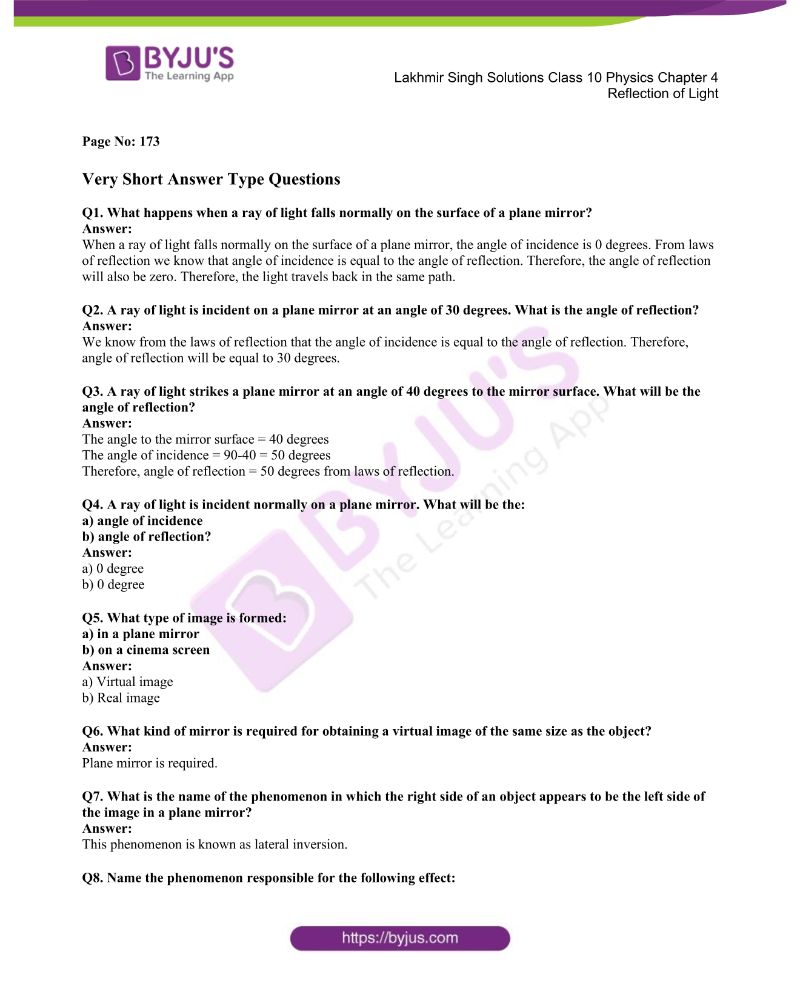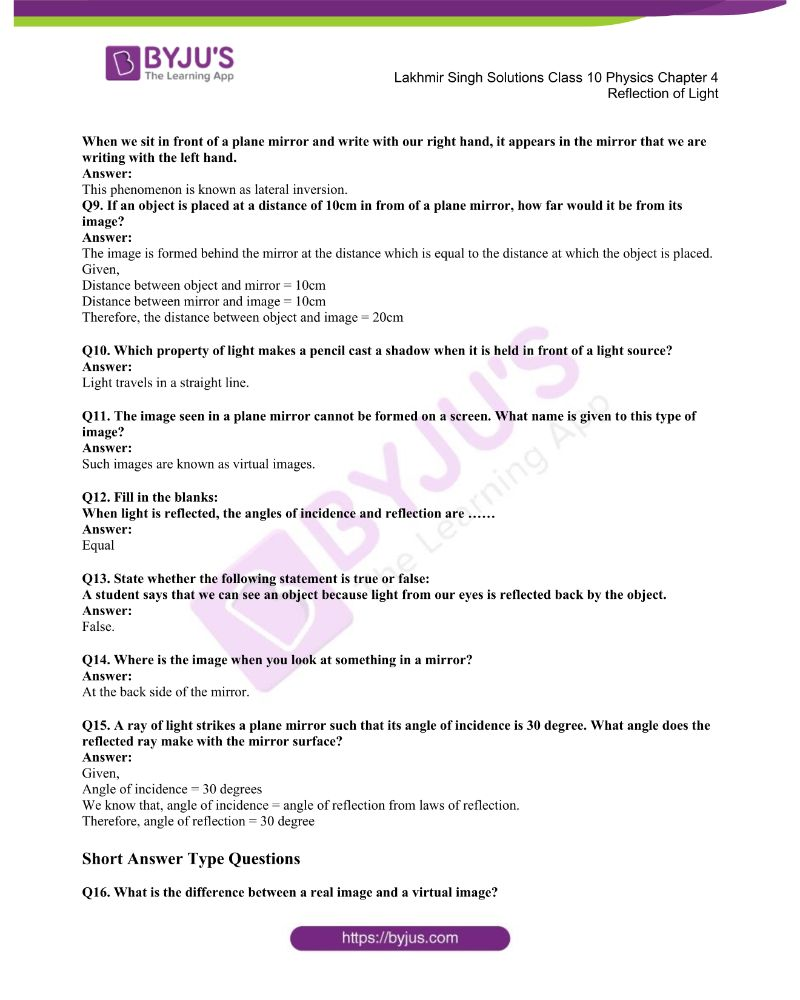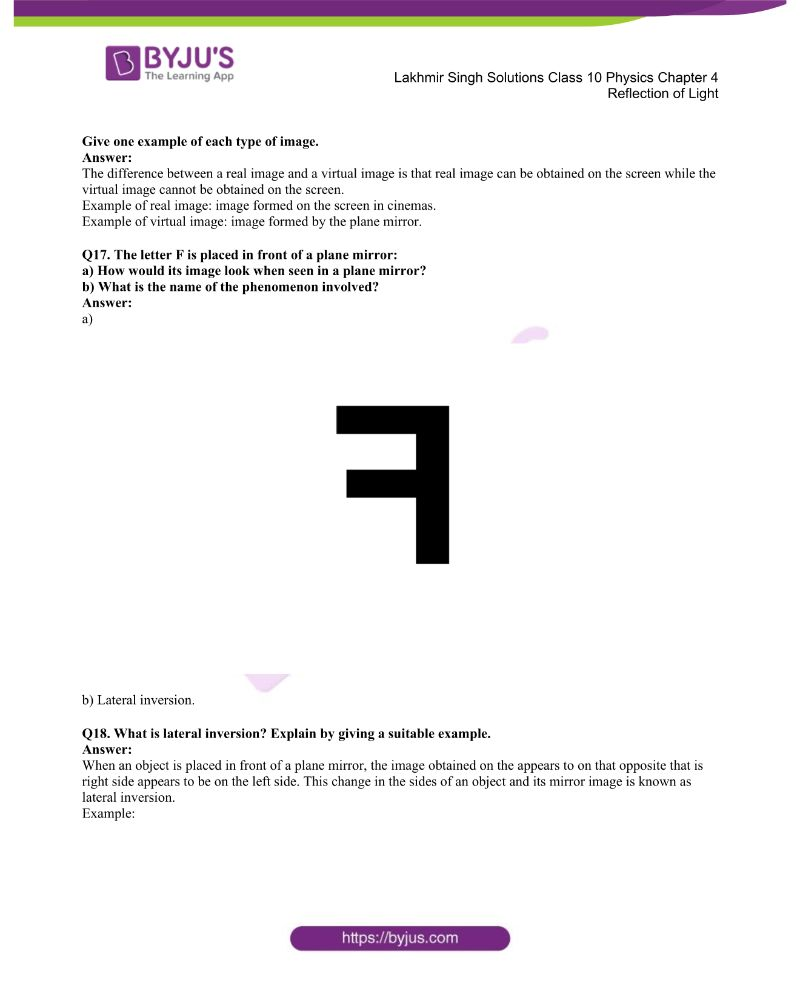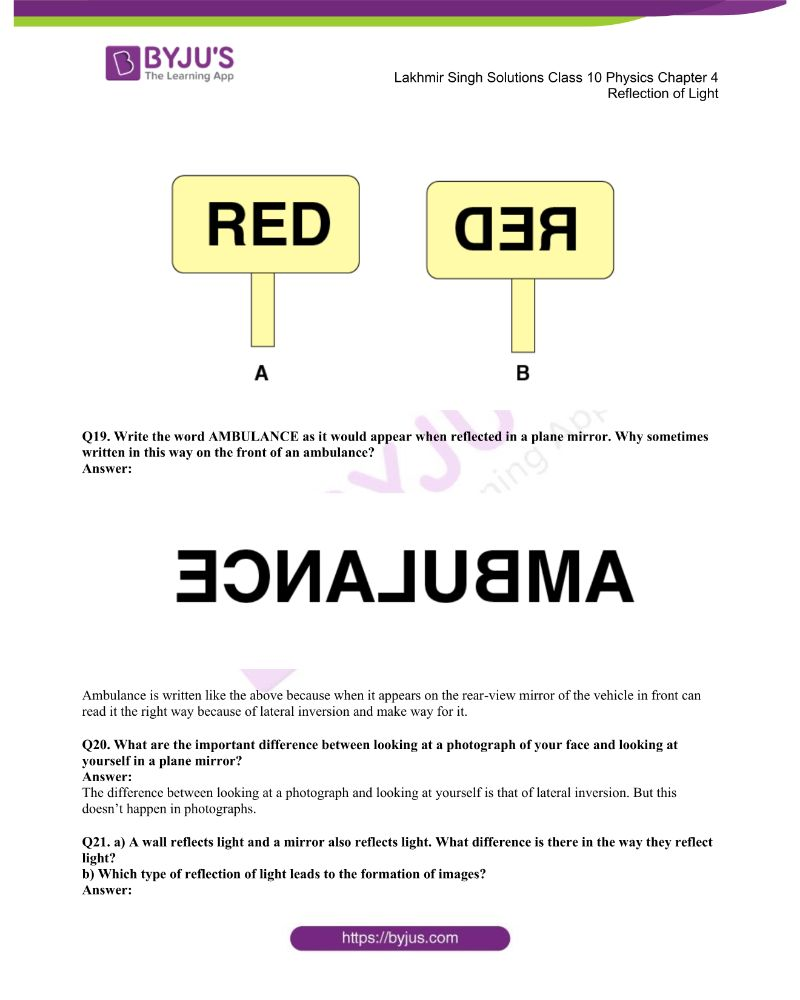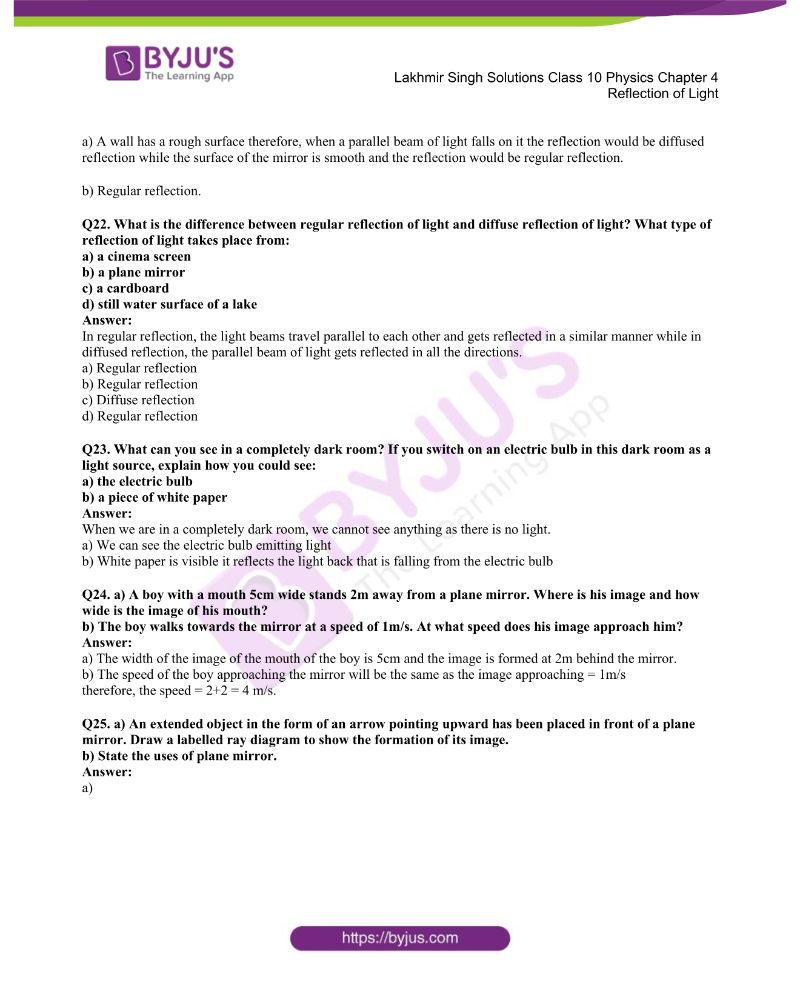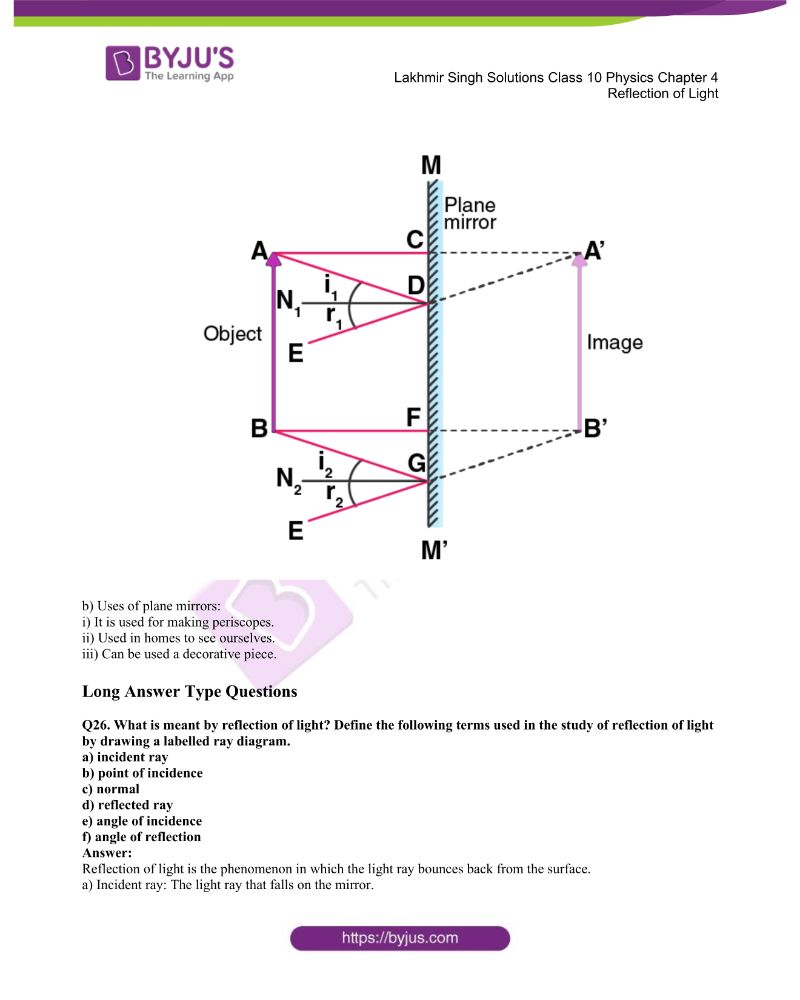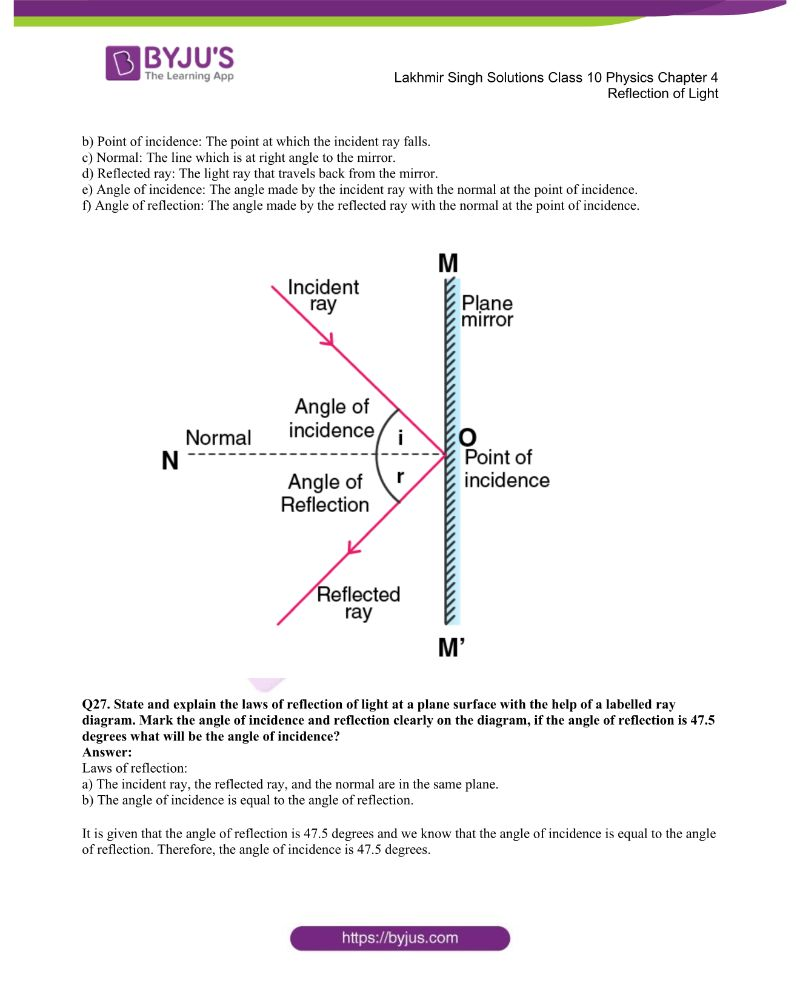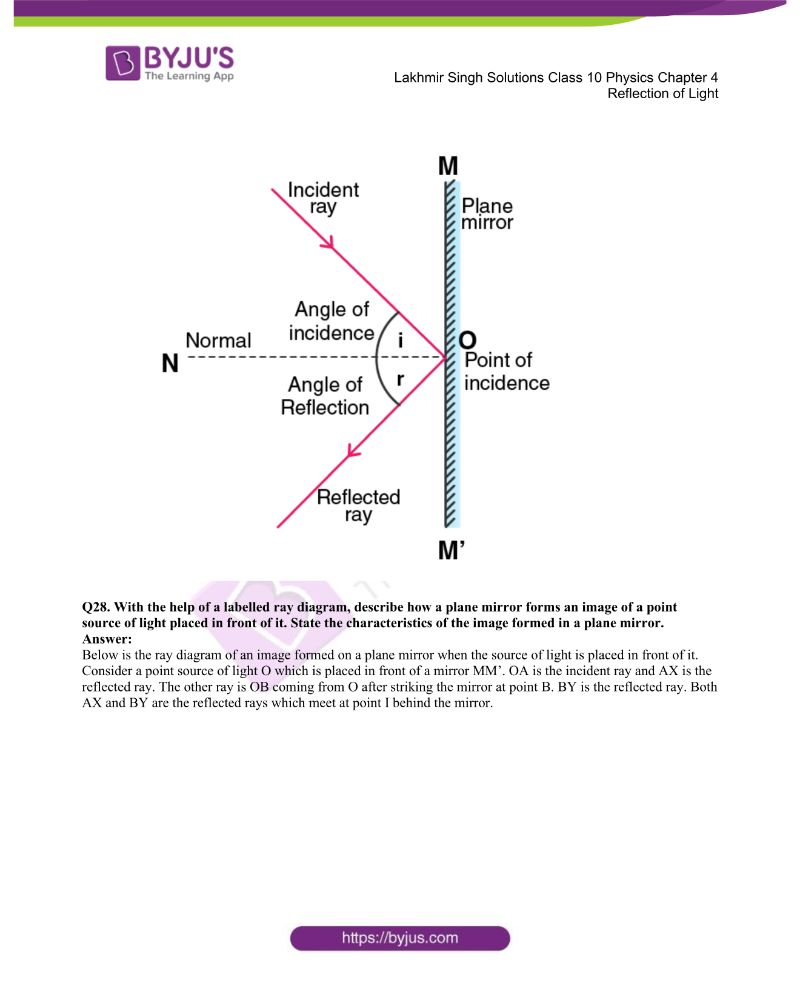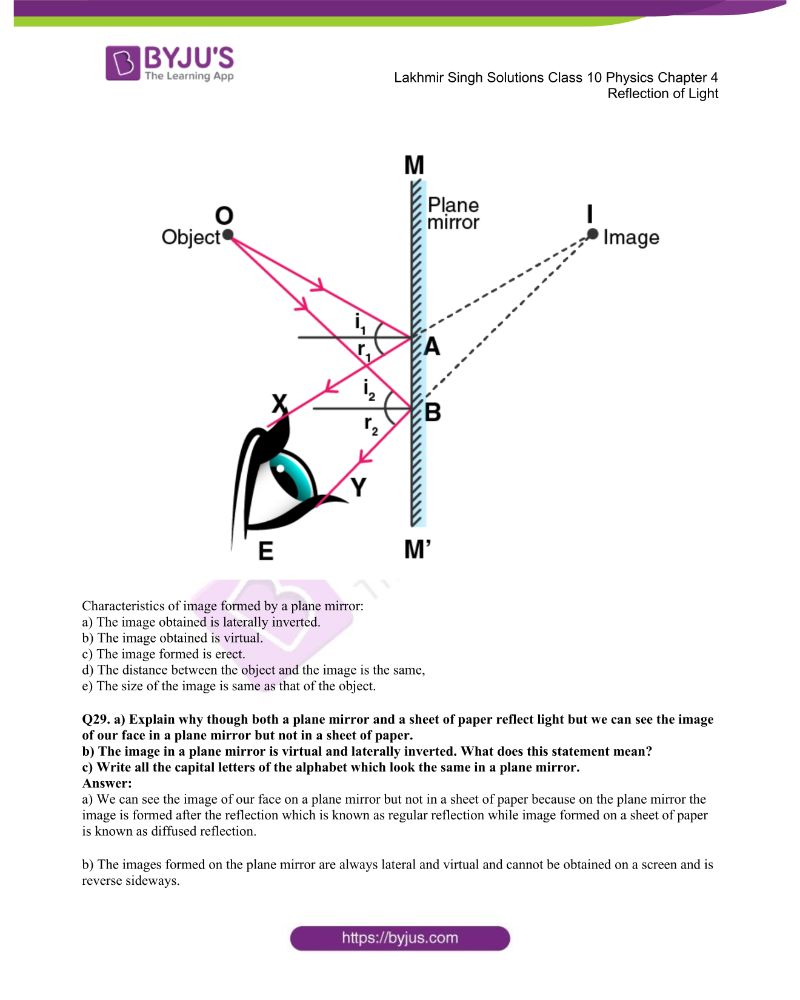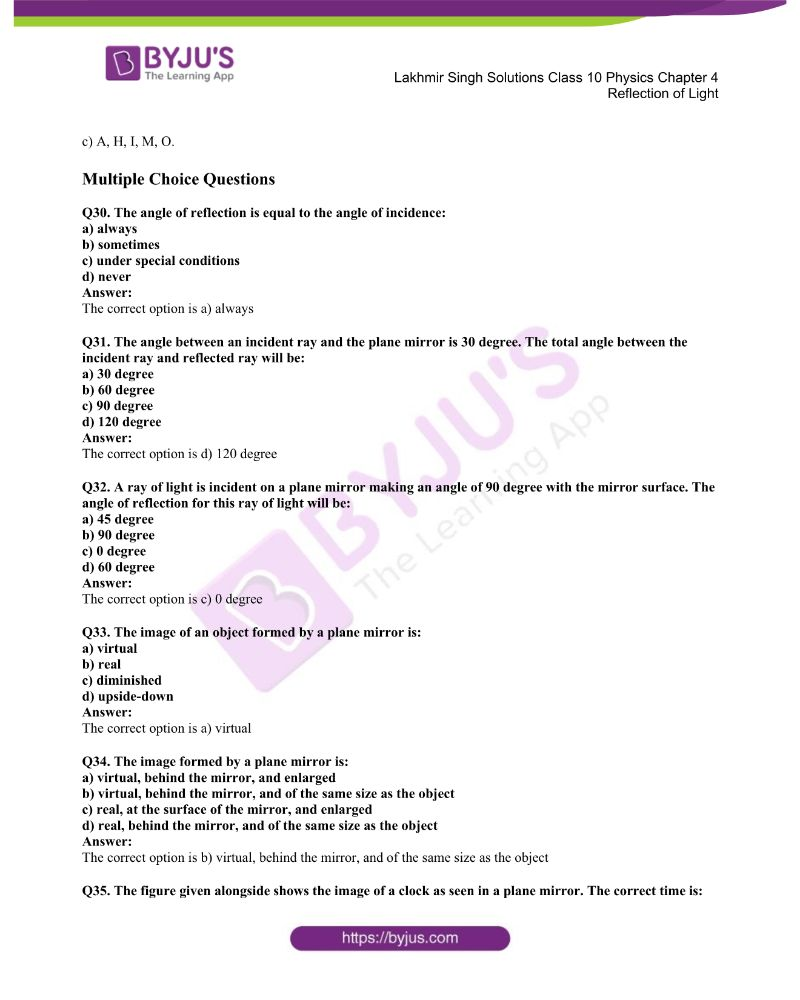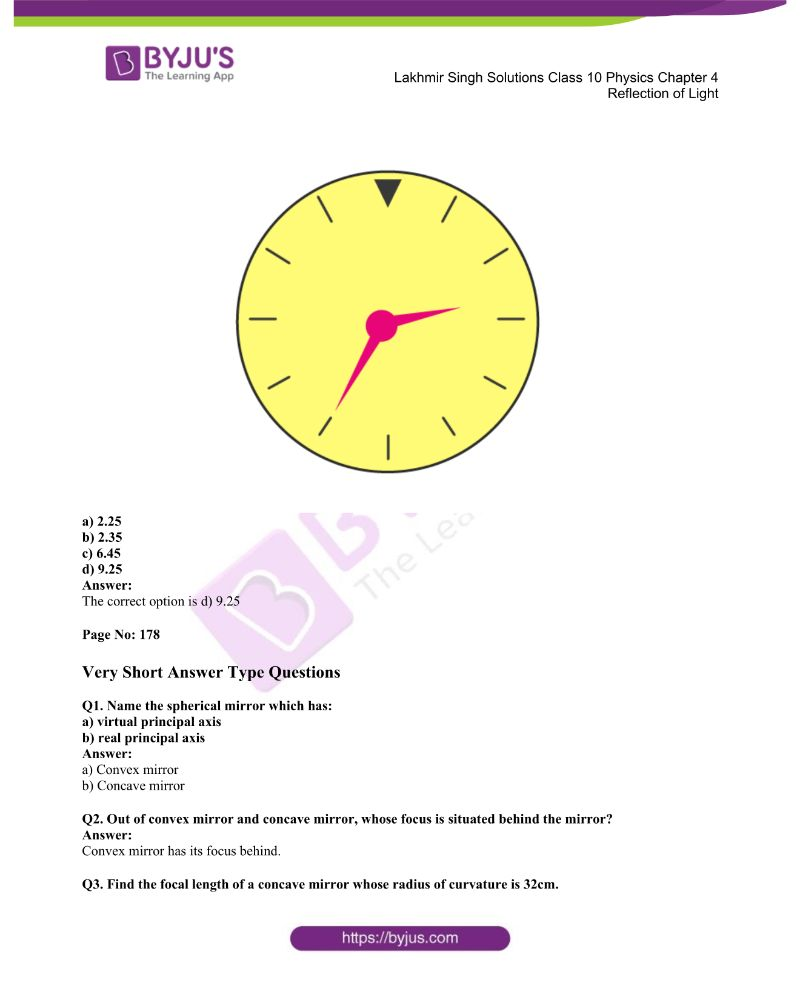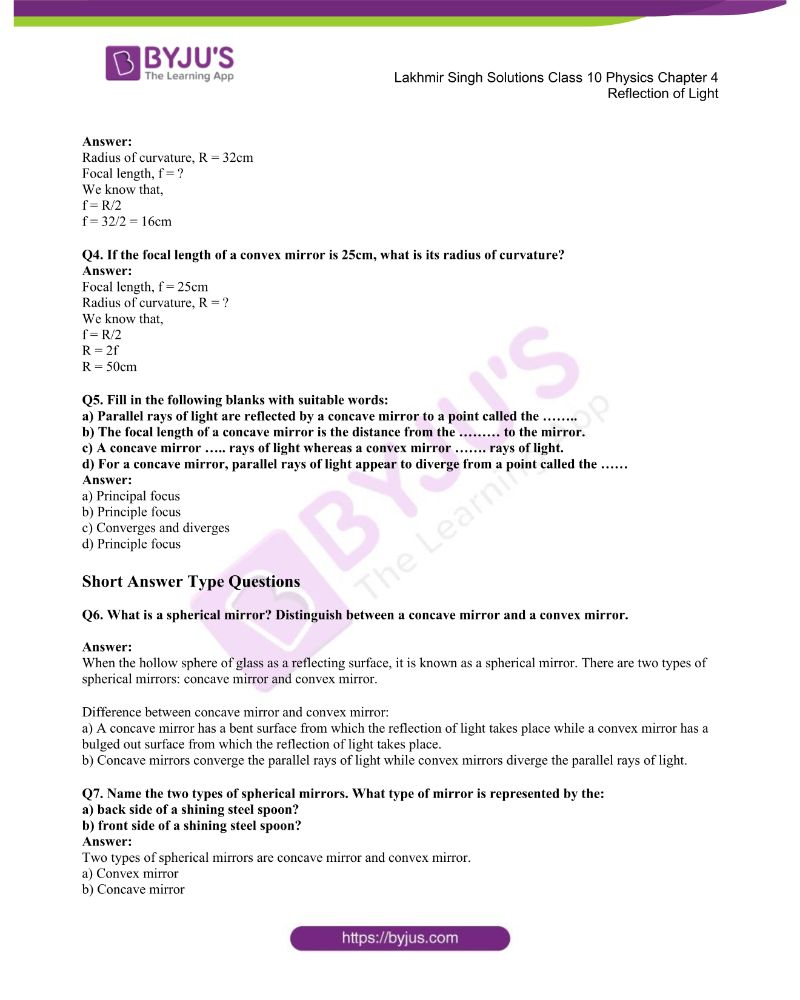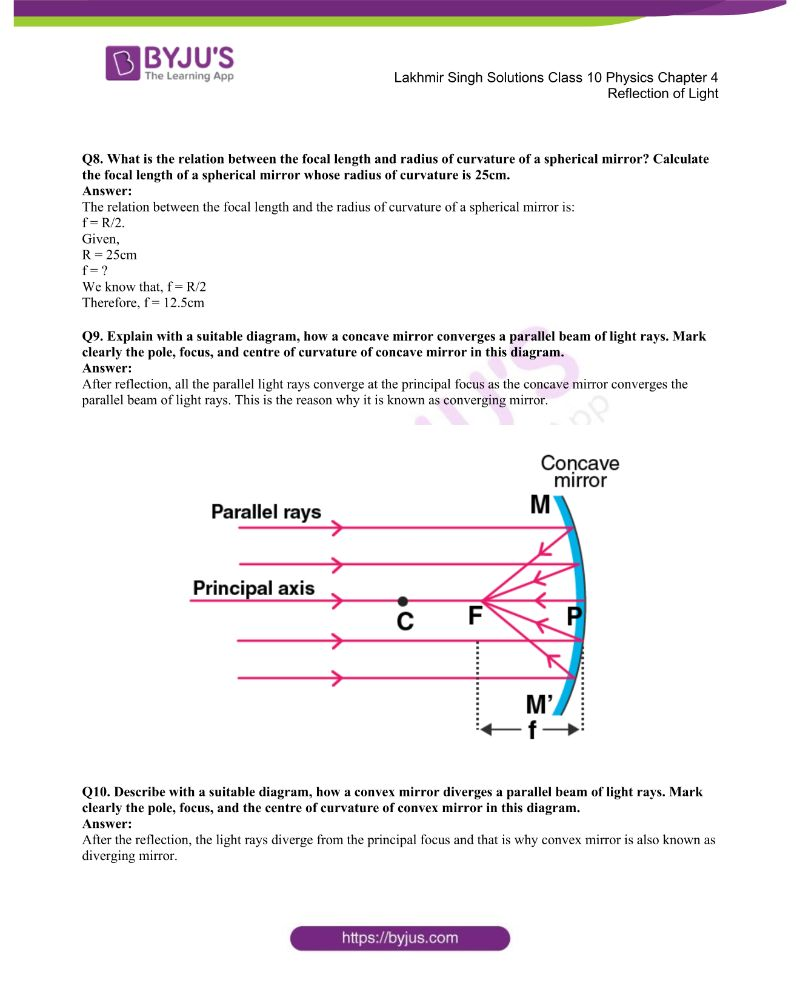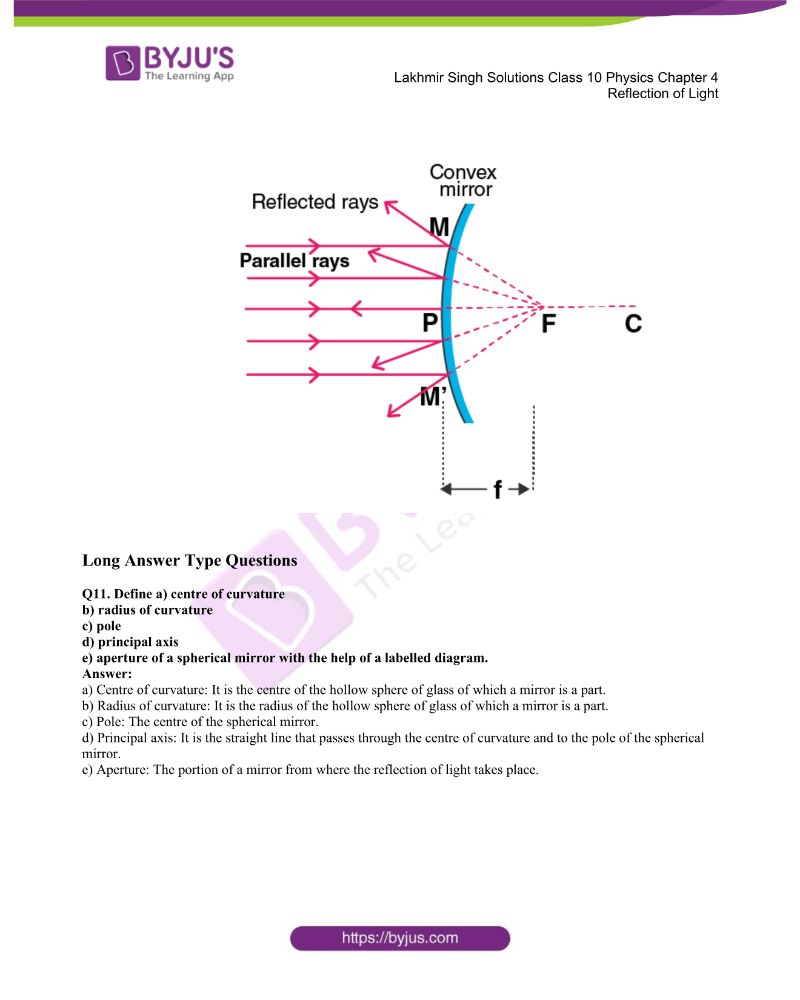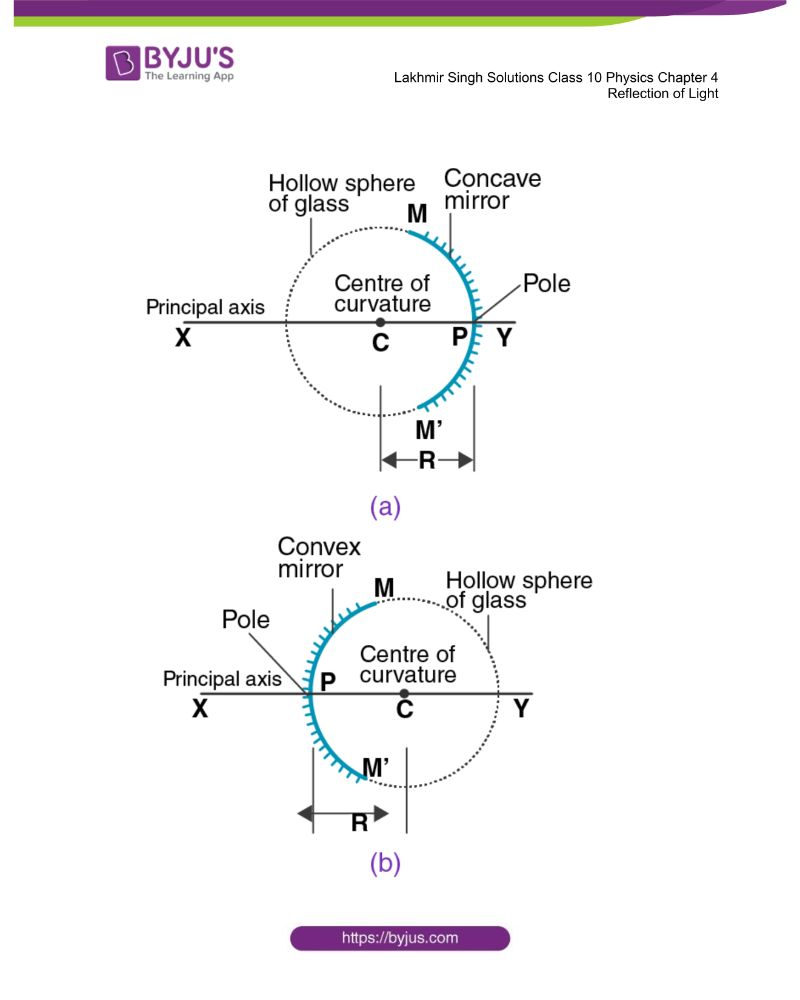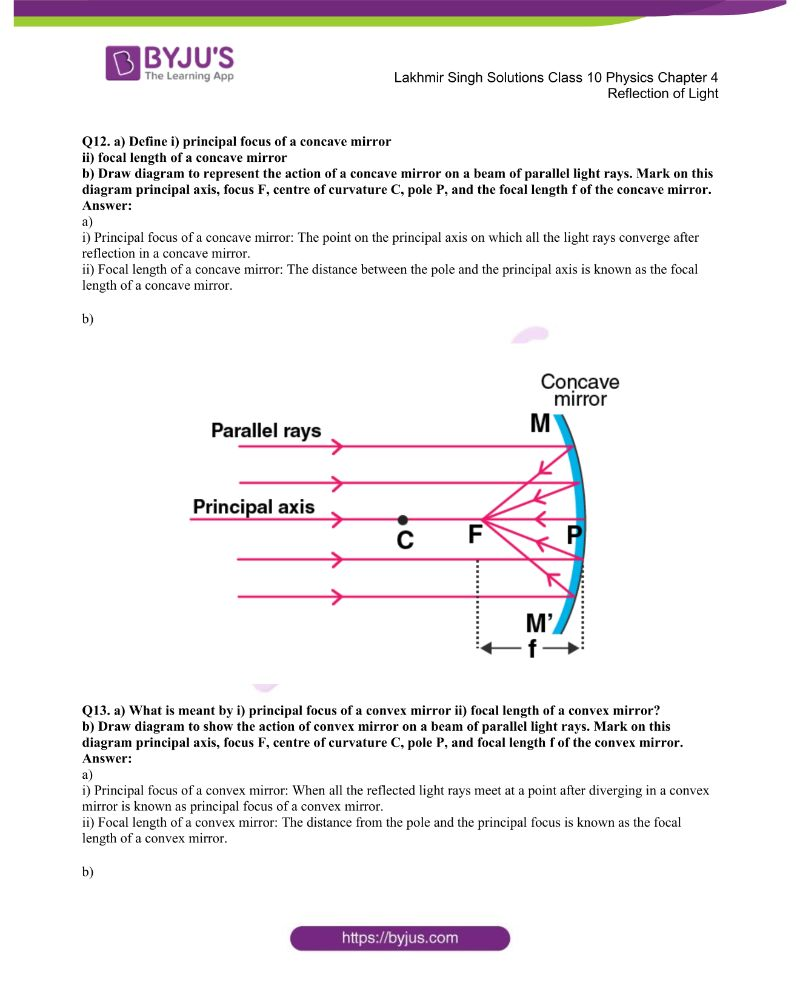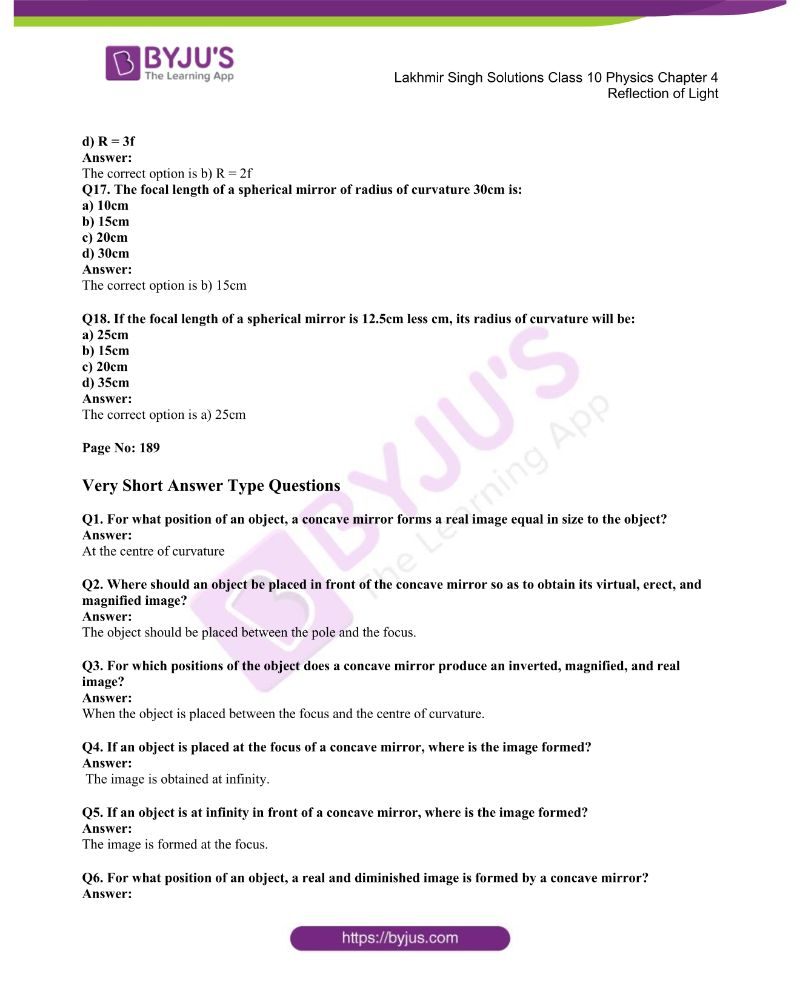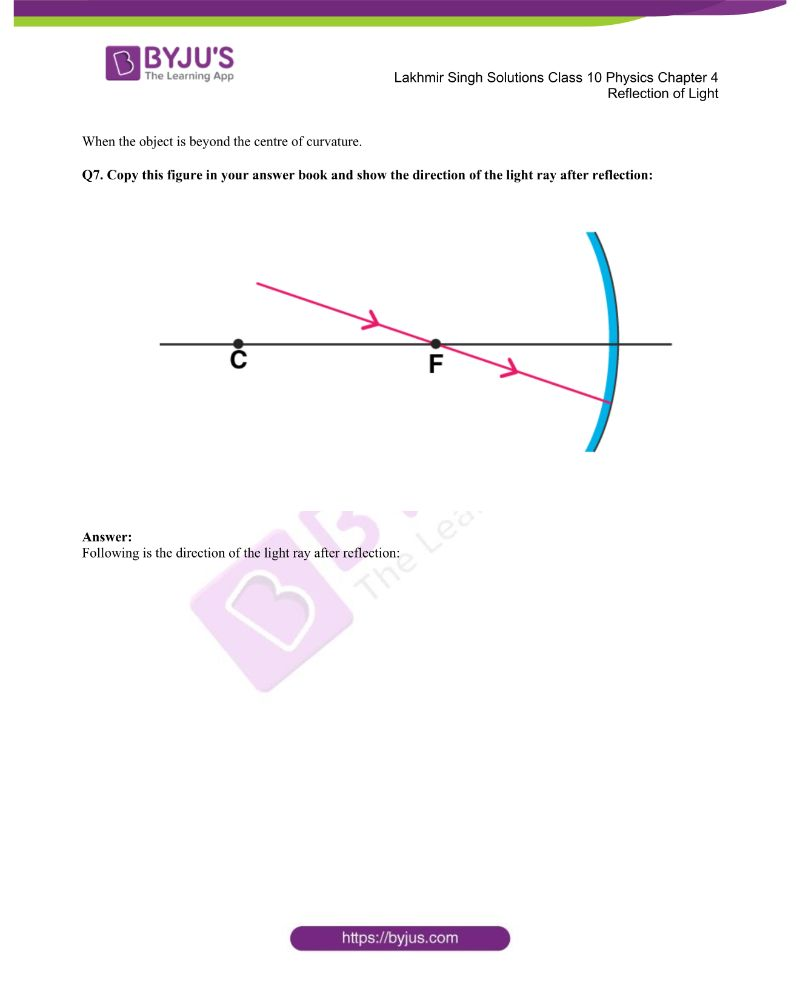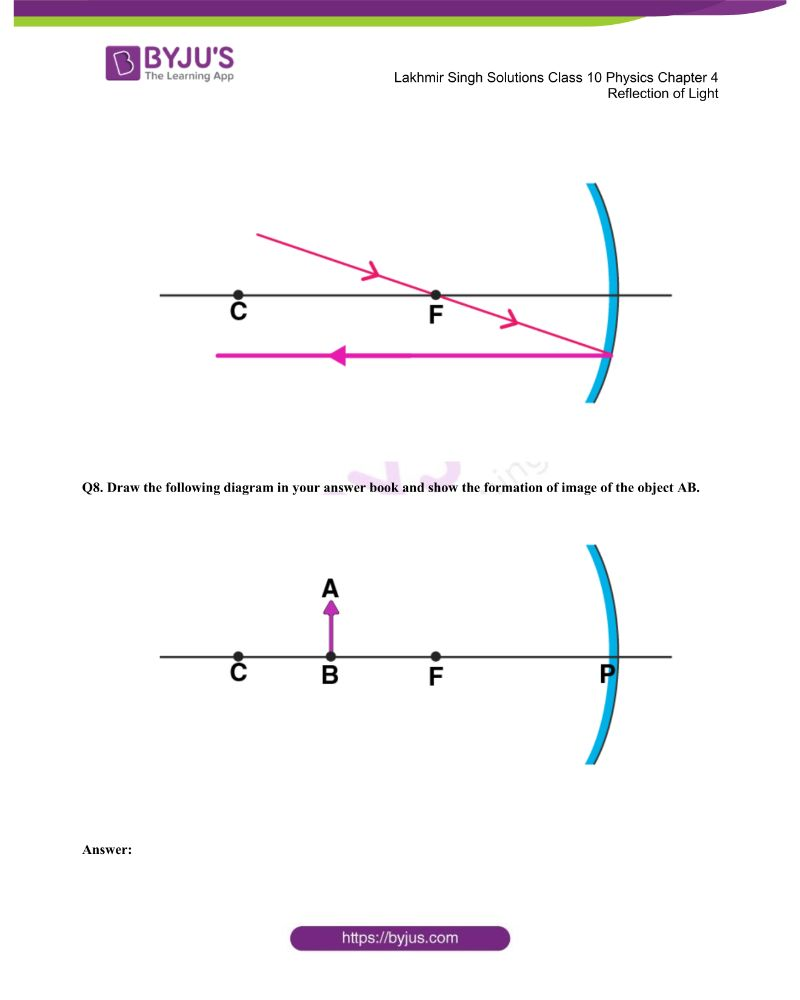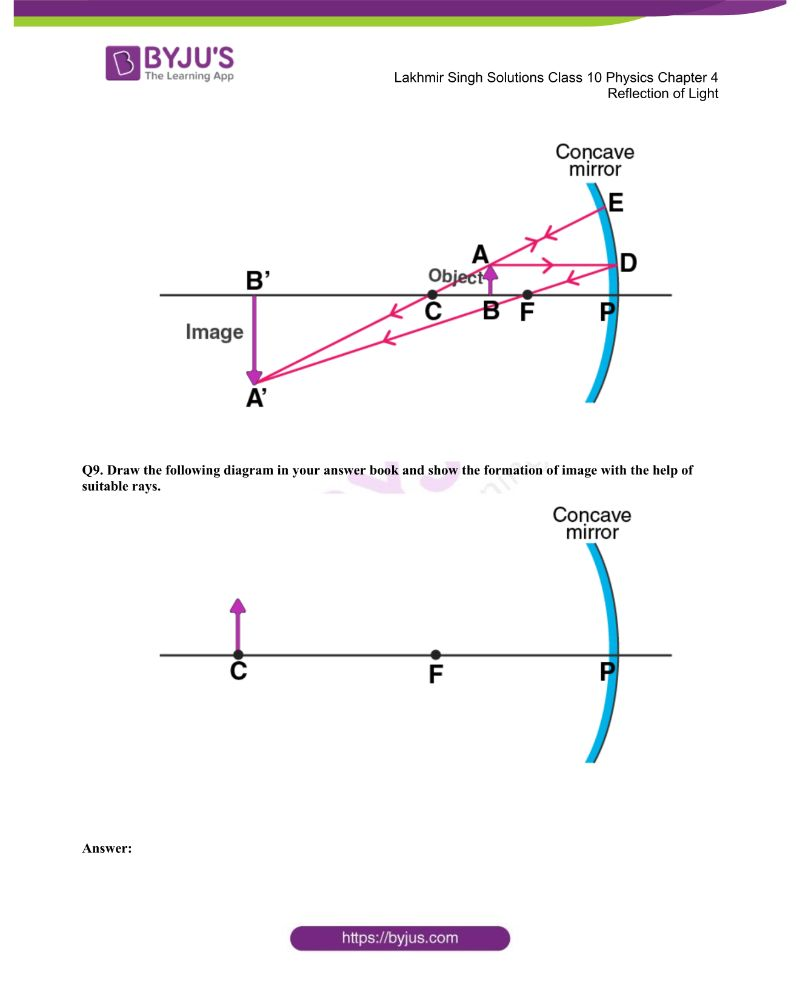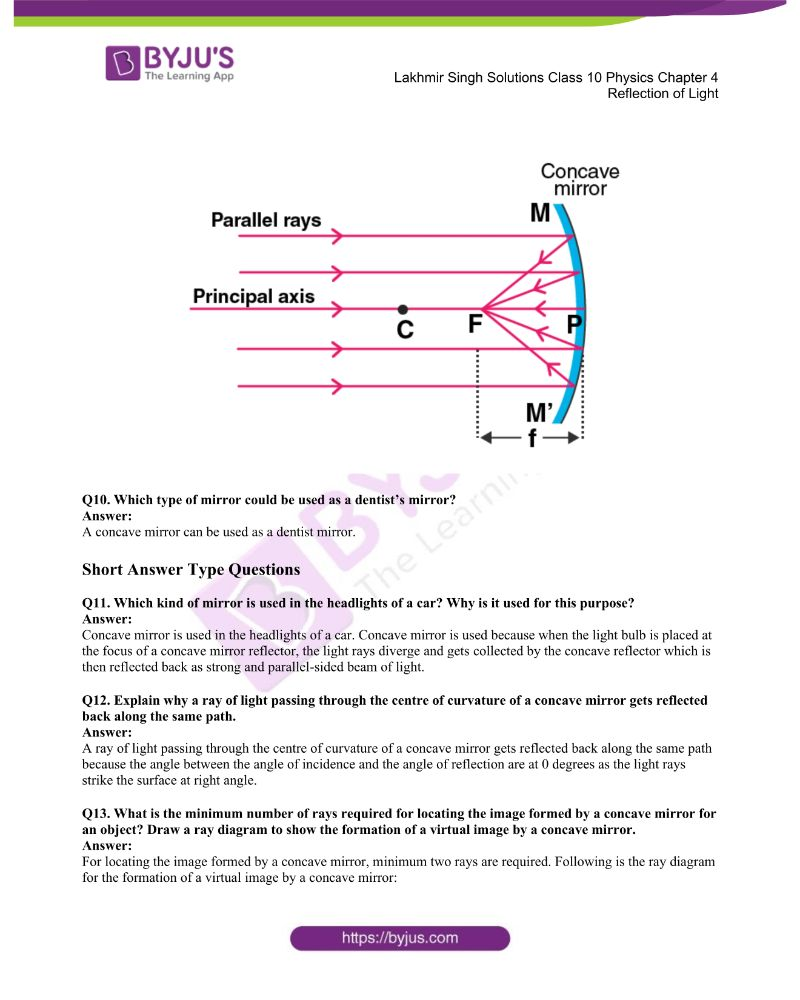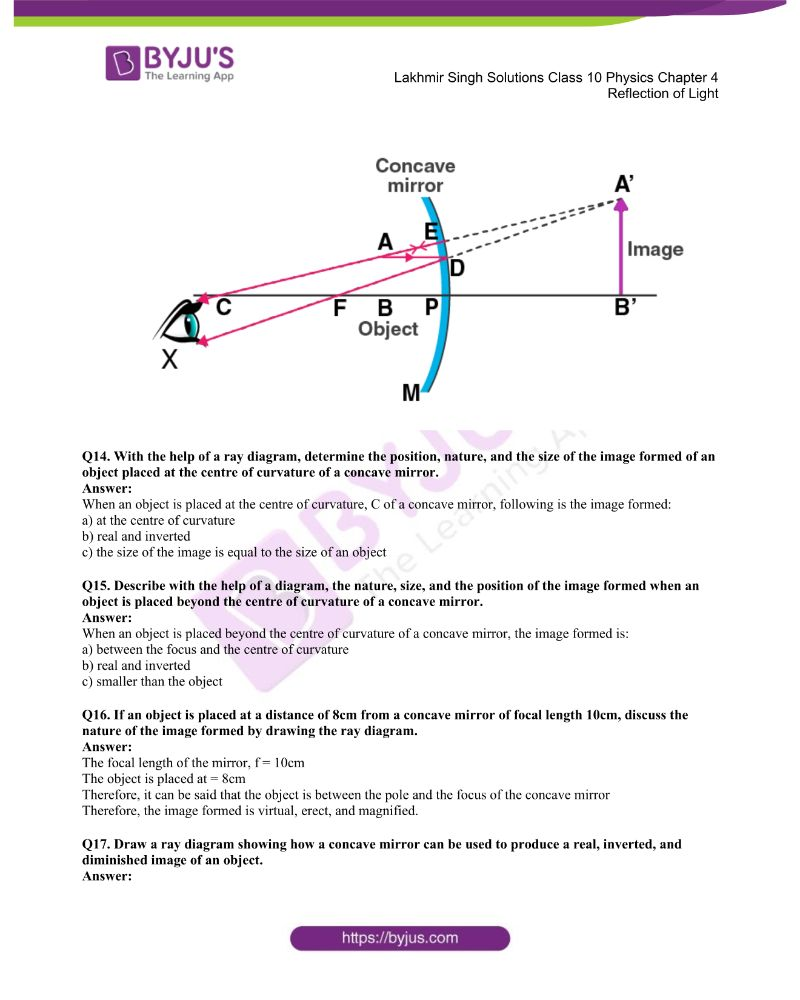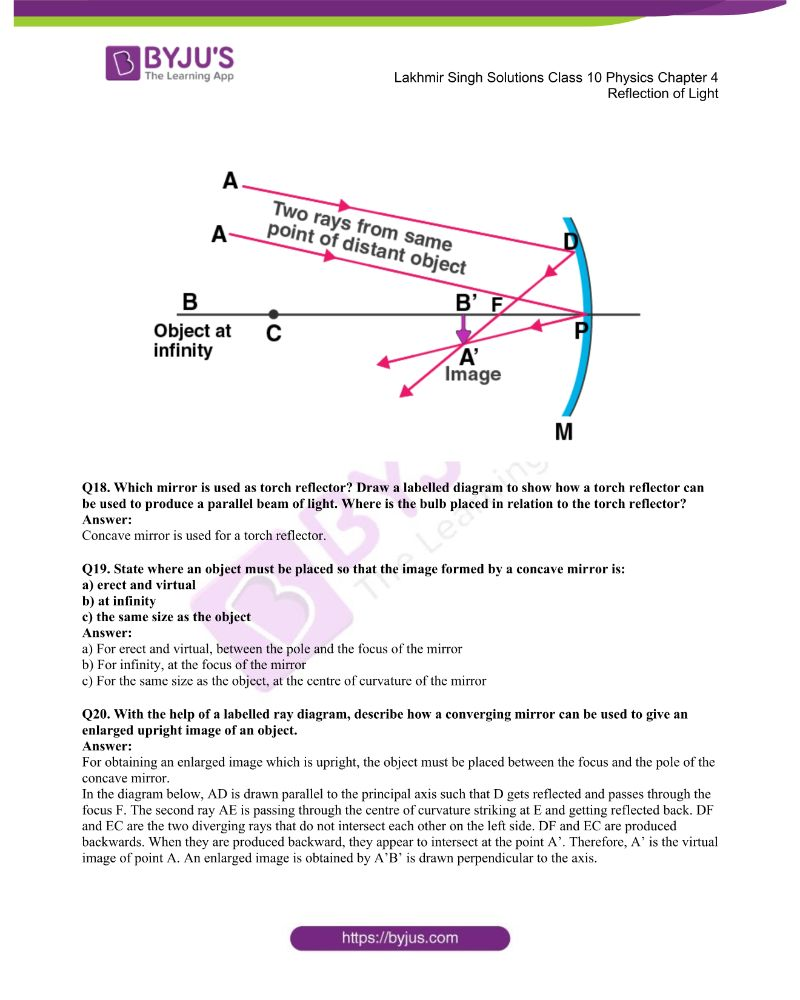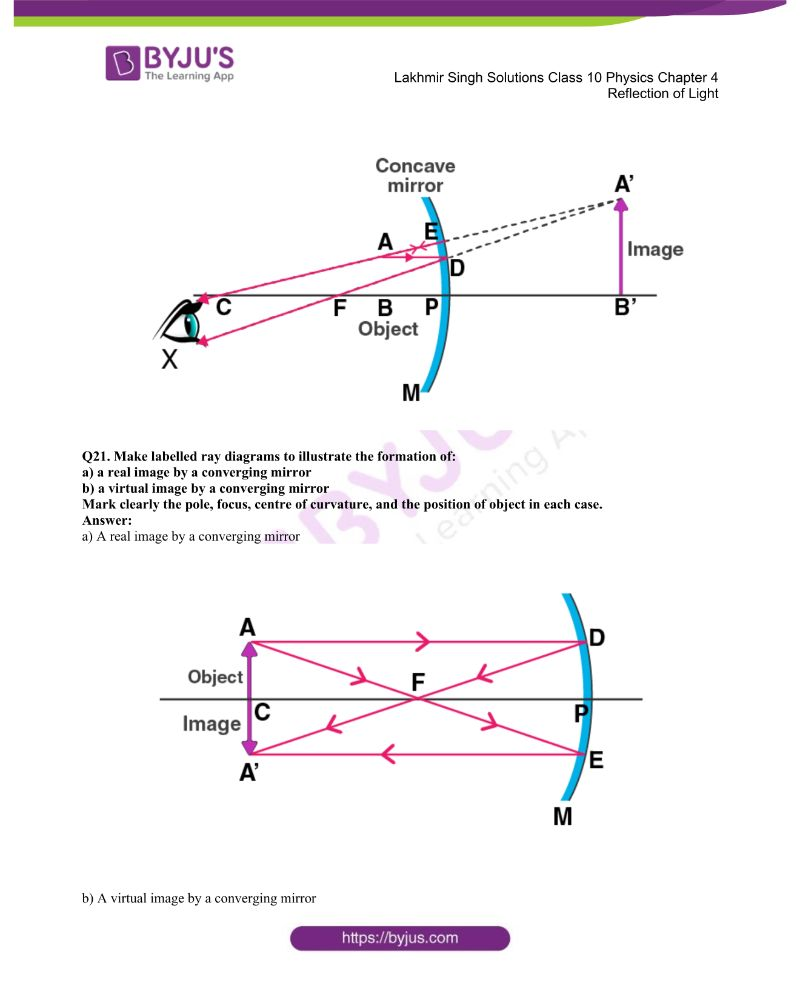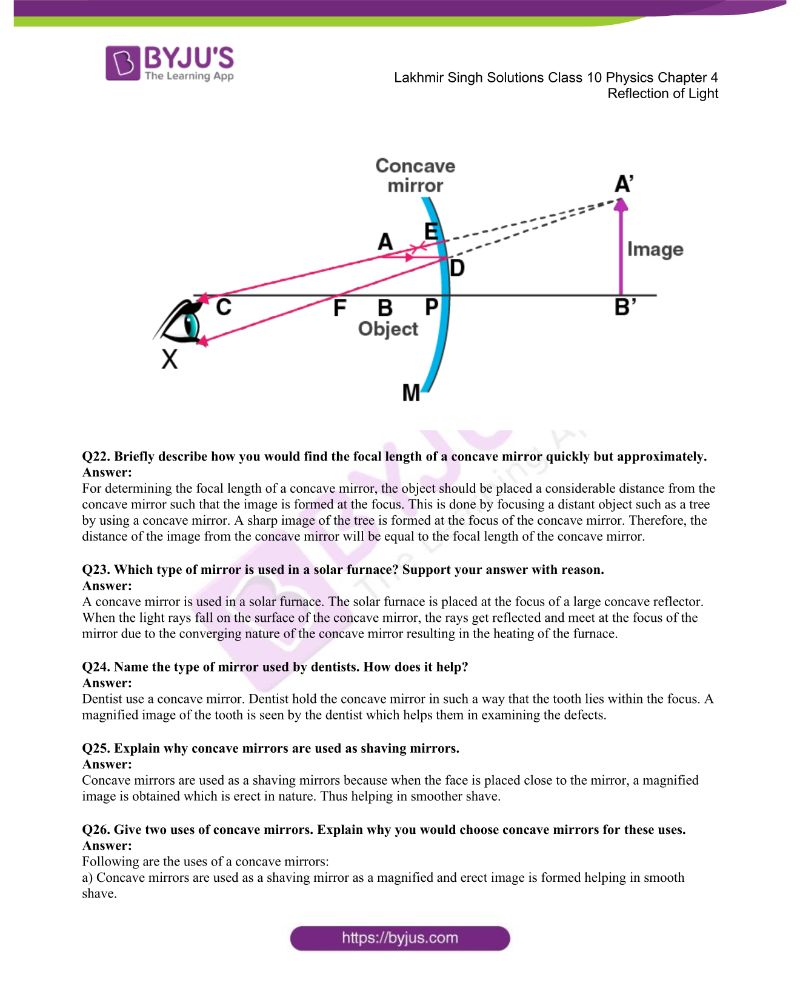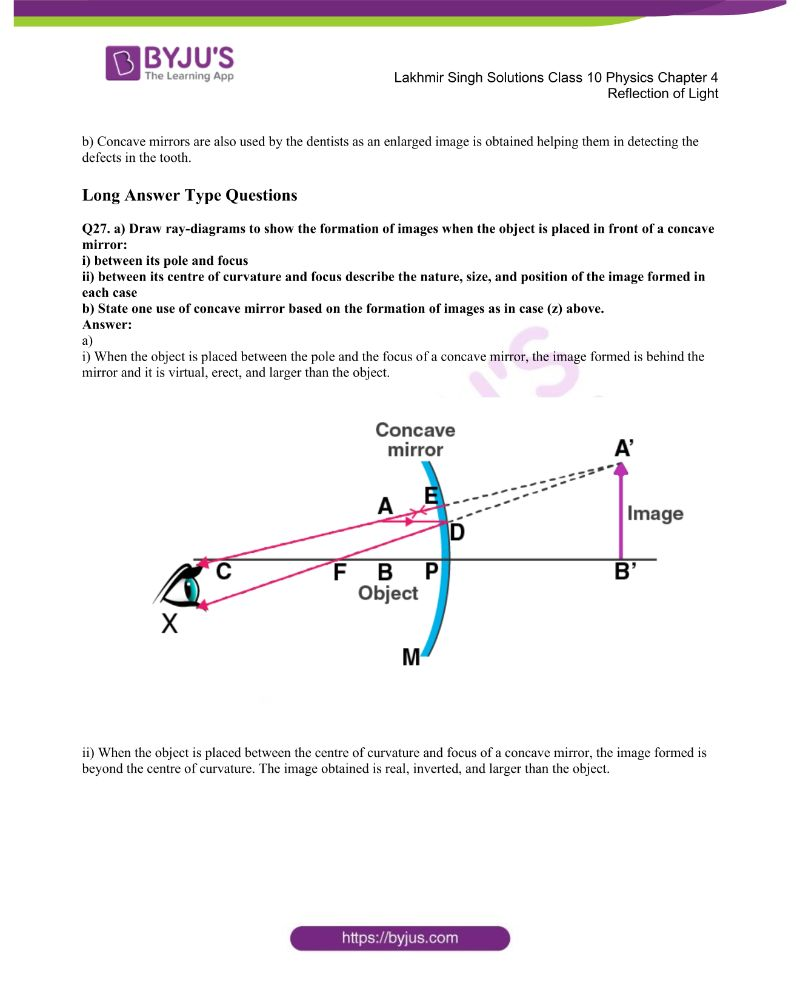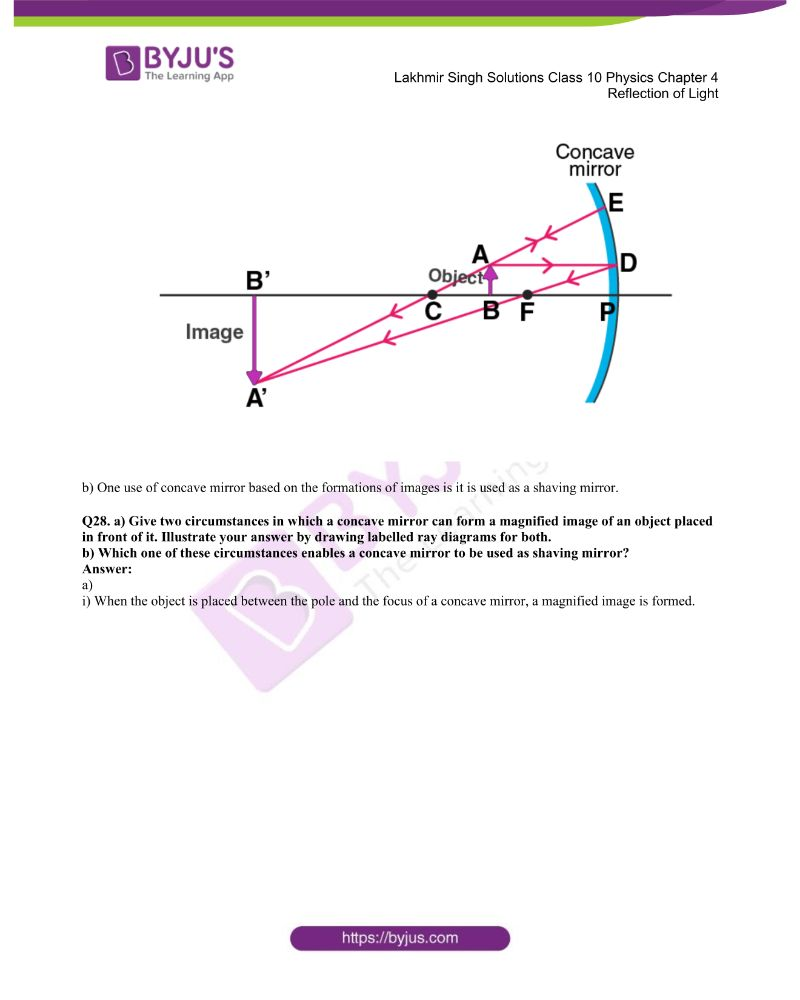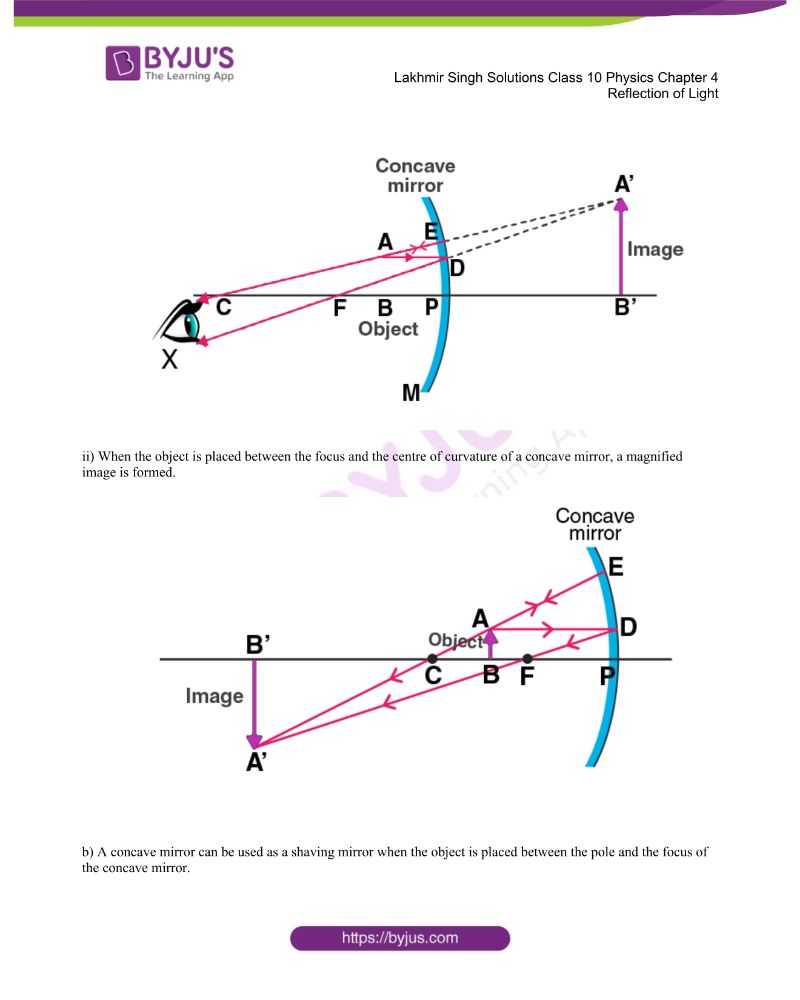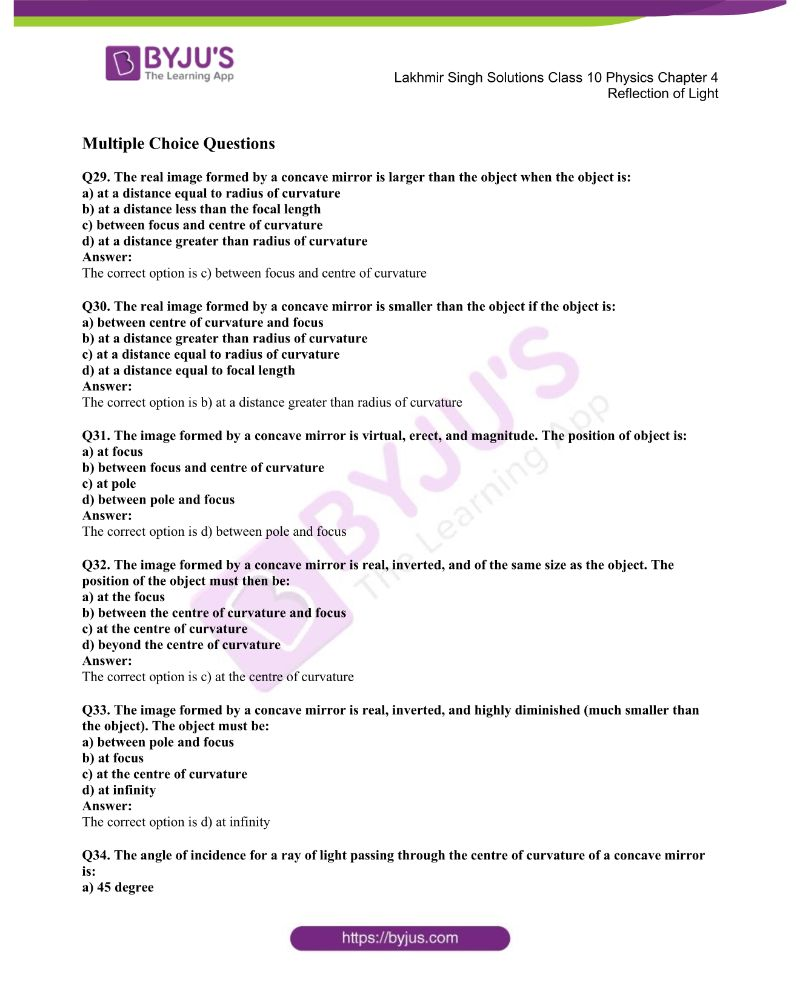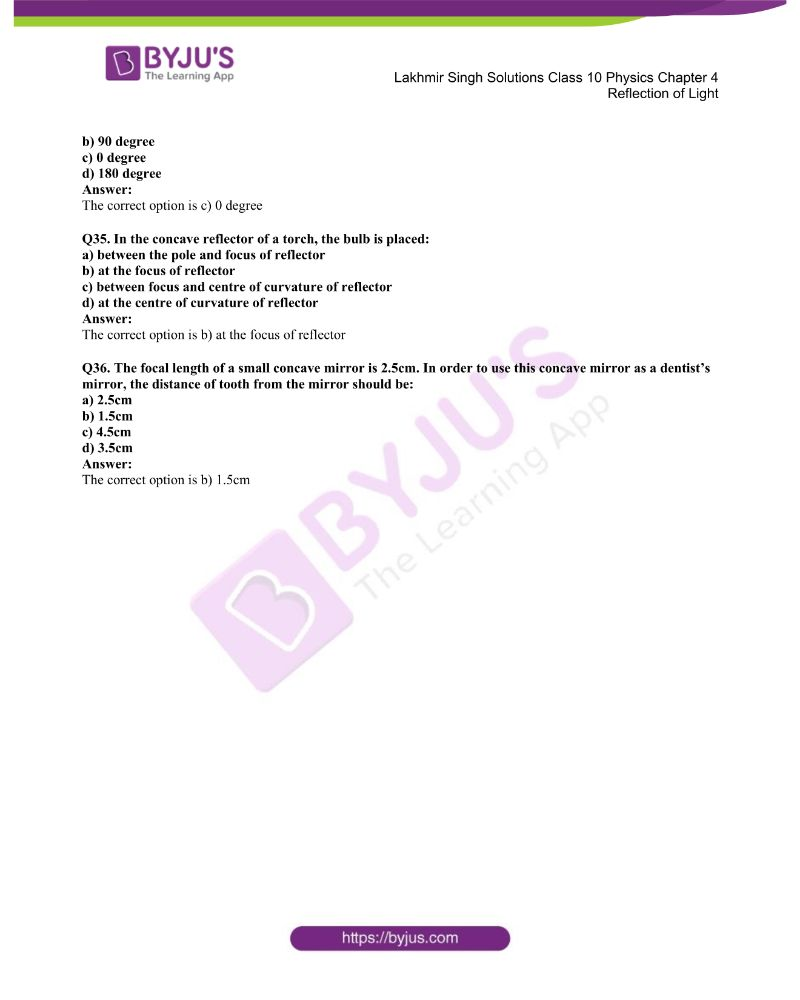### Access Lakhmir Singh Solutions Class 10 Physics Chapter 4 Solutions

Page No: 173

Q1. What happens when a ray of light falls normally on the surface of a plane mirror?

When a ray of light falls normally on the surface of a plane mirror, the angle of incidence is 0 degrees. From laws of reflection we know that angle of incidence is equal to the angle of reflection. Therefore, the angle of reflection will also be zero. Therefore, the light travels back in the same path.

Q2. A ray of light is incident on a plane mirror at an angle of 30 degrees. What is the angle of reflection?

We know from the laws of reflection that the angle of incidence is equal to the angle of reflection. Therefore, angle of reflection will be equal to 30 degrees.

Q3. A ray of light strikes a plane mirror at an angle of 40 degrees to the mirror surface. What will be the angle of reflection?

The angle to the mirror surface = 40 degrees

The angle of incidence = 90-40 = 50 degrees

Therefore, angle of reflection = 50 degrees from laws of reflection.

Q4. A ray of light is incident normally on a plane mirror. What will be the:

a) angle of incidence

b) angle of reflection?

a) 0 degree

b) 0 degree

Q5. What type of image is formed:

a) in a plane mirror

b) on a cinema screen

a) Virtual image

b) Real image

Q6. What kind of mirror is required for obtaining a virtual image of the same size as the object?

Plane mirror is required.

Q7. What is the name of the phenomenon in which the right side of an object appears to be the left side of the image in a plane mirror?

This phenomenon is known as lateral inversion.

Q8. Name the phenomenon responsible for the following effect:

When we sit in front of a plane mirror and write with our right hand, it appears in the mirror that we are writing with the left hand.

This phenomenon is known as lateral inversion.

Q9. If an object is placed at a distance of 10cm in from of a plane mirror, how far would it be from its image?

The image is formed behind the mirror at the distance which is equal to the distance at which the object is placed.

Given,

Distance between object and mirror = 10cm

Distance between mirror and image = 10cm

Therefore, the distance between object and image = 20cm

Q10. Which property of light makes a pencil cast a shadow when it is held in front of a light source?

Light travels in a straight line.

Q11. The image seen in a plane mirror cannot be formed on a screen. What name is given to this type of image?

Such images are known as virtual images.

Q12. Fill in the blanks:

When light is reflected, the angles of incidence and reflection are ……

Equal

Q13. State whether the following statement is true or false:

A student says that we can see an object because light from our eyes is reflected back by the object.

False.

Q14. Where is the image when you look at something in a mirror?

At the back side of the mirror.

Q15. A ray of light strikes a plane mirror such that its angle of incidence is 30 degree. What angle does the reflected ray make with the mirror surface?

Given,

Angle of incidence = 30 degrees

We know that, angle of incidence = angle of reflection from laws of reflection.

Therefore, angle of reflection = 30 degree

Q16. What is the difference between a real image and a virtual image?

Give one example of each type of image.

The difference between a real image and a virtual image is that real image can be obtained on the screen while the virtual image cannot be obtained on the screen.

Example of real image: image formed on the screen in cinemas.

Example of virtual image: image formed by the plane mirror.

Q17. The letter F is placed in front of a plane mirror:

a) How would its image look when seen in a plane mirror?

b) What is the name of the phenomenon involved?

a)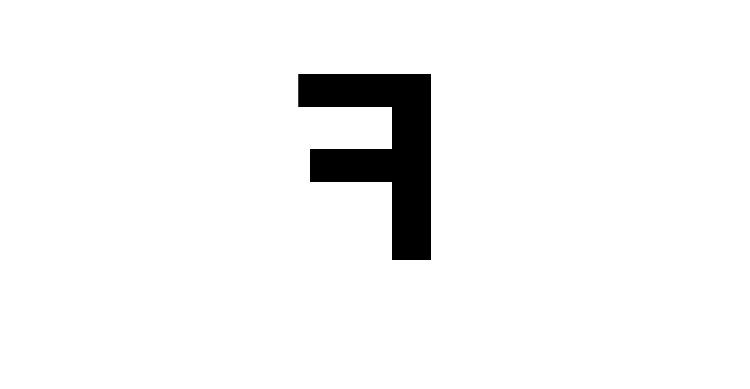b) Lateral inversion.

Q18. What is lateral inversion? Explain by giving a suitable example.

When an object is placed in front of a plane mirror, the image obtained on the appears to on that opposite that is right side appears to be on the left side. This change in the sides of an object and its mirror image is known as lateral inversion.

Example: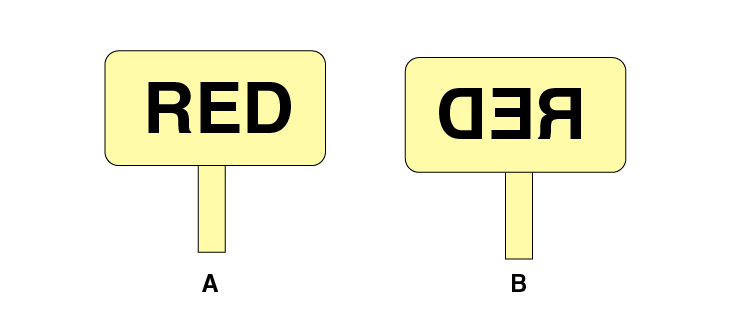Q19. Write the word AMBULANCE as it would appear when reflected in a plane mirror. Why sometimes written in this way on the front of an ambulance?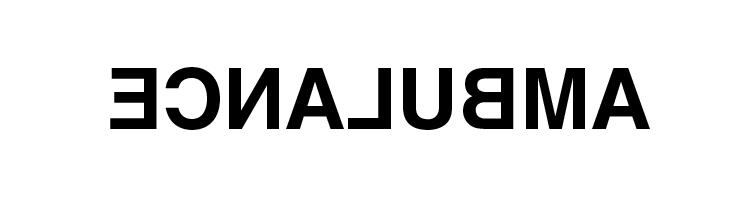Ambulance is written like the above because when it appears on the rear-view mirror of the vehicle in front can read it the right way because of lateral inversion and make way for it.

Q20. What are the important difference between looking at a photograph of your face and looking at yourself in a plane mirror?

The difference between looking at a photograph and looking at yourself is that of lateral inversion. But this doesn’t happen in photographs.

Q21. a) A wall reflects light and a mirror also reflects light. What difference is there in the way they reflect light?

b) Which type of reflection of light leads to the formation of images?

a) A wall has a rough surface therefore, when a parallel beam of light falls on it the reflection would be diffused reflection while the surface of the mirror is smooth and the reflection would be regular reflection.

b) Regular reflection.

Q22. What is the difference between regular reflection of light and diffuse reflection of light? What type of reflection of light takes place from:

a) a cinema screen

b) a plane mirror

c) a cardboard

d) still water surface of a lake

In regular reflection, the light beams travel parallel to each other and gets reflected in a similar manner while in diffused reflection, the parallel beam of light gets reflected in all the directions.

a) Regular reflection

b) Regular reflection

c) Diffuse reflection

d) Regular reflection

Q23. What can you see in a completely dark room? If you switch on an electric bulb in this dark room as a light source, explain how you could see:

a) the electric bulb

b) a piece of white paper

When we are in a completely dark room, we cannot see anything as there is no light.

a) We can see the electric bulb emitting light

b) White paper is visible it reflects the light back that is falling from the electric bulb

Q24. a) A boy with a mouth 5cm wide stands 2m away from a plane mirror. Where is his image and how wide is the image of his mouth?

b) The boy walks towards the mirror at a speed of 1m/s. At what speed does his image approach him?

a) The width of the image of the mouth of the boy is 5cm and the image is formed at 2m behind the mirror.

b) The speed of the boy approaching the mirror will be the same as the image approaching = 1m/s

therefore, the speed = 2+2 = 4 m/s.

Q25. a) An extended object in the form of an arrow pointing upward has been placed in front of a plane mirror. Draw a labelled ray diagram to show the formation of its image.

b) State the uses of plane mirror.

a)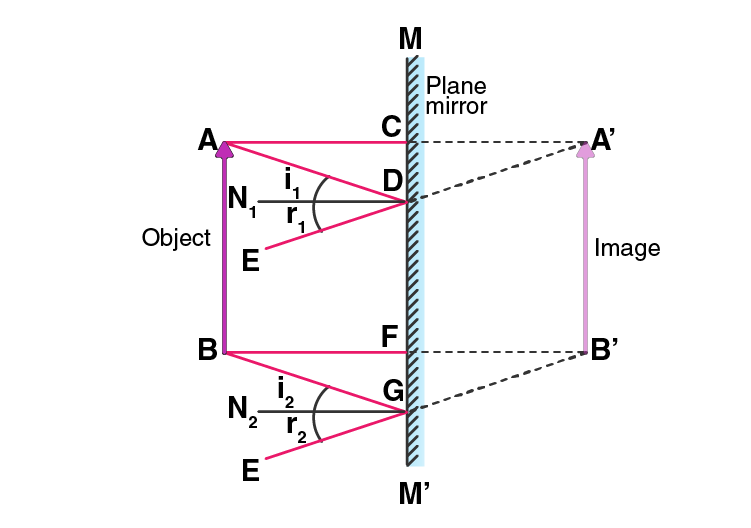b) Uses of plane mirrors:

i) It is used for making periscopes.

ii) Used in homes to see ourselves.

iii) Can be used a decorative piece.

Q26. What is meant by reflection of light? Define the following terms used in the study of reflection of light by drawing a labelled ray diagram.

a) incident ray

b) point of incidence

c) normal

d) reflected ray

e) angle of incidence

f) angle of reflection

Reflection of light is the phenomenon in which the light ray bounces back from the surface.

a) Incident ray: The light ray that falls on the mirror.

b) Point of incidence: The point at which the incident ray falls.

c) Normal: The line which is at right angle to the mirror.

d) Reflected ray: The light ray that travels back from the mirror.

e) Angle of incidence: The angle made by the incident ray with the normal at the point of incidence.

f) Angle of reflection: The angle made by the reflected ray with the normal at the point of incidence.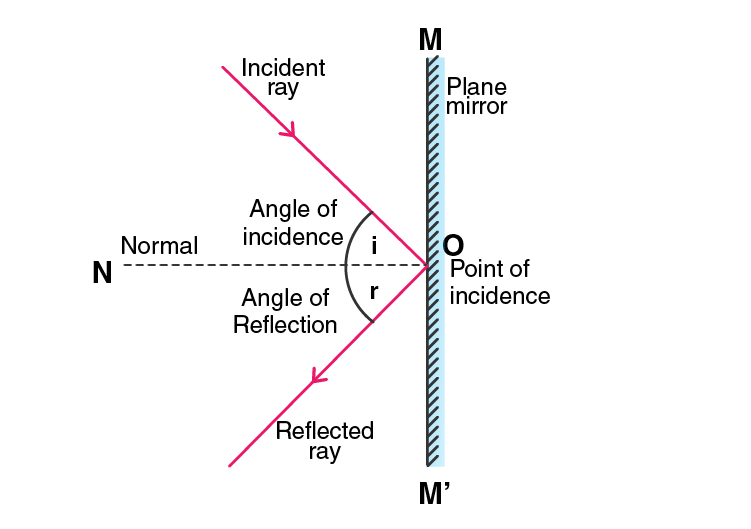Q27. State and explain the laws of reflection of light at a plane surface with the help of a labelled ray diagram. Mark the angle of incidence and reflection clearly on the diagram, if the angle of reflection is 47.5 degrees what will be the angle of incidence?

Laws of reflection:

a) The incident ray, the reflected ray, and the normal are in the same plane.

b) The angle of incidence is equal to the angle of reflection.

It is given that the angle of reflection is 47.5 degrees and we know that the angle of incidence is equal to the angle of reflection. Therefore, the angle of incidence is 47.5 degrees.Q28. With the help of a labelled ray diagram, describe how a plane mirror forms an image of a point source of light placed in front of it. State the characteristics of the image formed in a plane mirror.

Below is the ray diagram of an image formed on a plane mirror when the source of light is placed in front of it. Consider a point source of light O which is placed in front of a mirror MM’. OA is the incident ray and AX is the reflected ray. The other ray is OB coming from O after striking the mirror at point B. BY is the reflected ray. Both AX and BY are the reflected rays which meet at point I behind the mirror.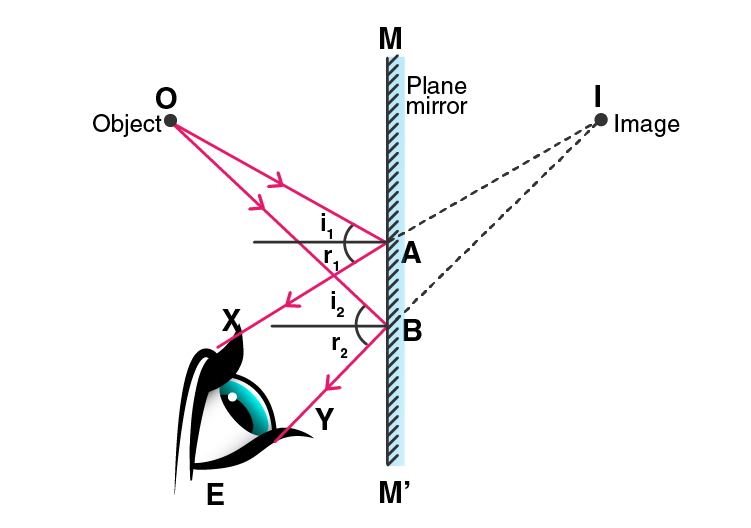Characteristics of image formed by a plane mirror:

a) The image obtained is laterally inverted.

b) The image obtained is virtual.

c) The image formed is erect.

d) The distance between the object and the image is the same,

e) The size of the image is same as that of the object.

Q29. a) Explain why though both a plane mirror and a sheet of paper reflect light but we can see the image of our face in a plane mirror but not in a sheet of paper.

b) The image in a plane mirror is virtual and laterally inverted. What does this statement mean?

c) Write all the capital letters of the alphabet which look the same in a plane mirror.

a) We can see the image of our face on a plane mirror but not in a sheet of paper because on the plane mirror the image is formed after the reflection which is known as regular reflection while image formed on a sheet of paper is known as diffused reflection.

b) The images formed on the plane mirror are always lateral and virtual and cannot be obtained on a screen and is reverse sideways.

c) A, H, I, M, O.

Multiple Choice Questions

Q30. The angle of reflection is equal to the angle of incidence:

a) always

b) sometimes

c) under special conditions

d) never

The correct option is a) always

Q31. The angle between an incident ray and the plane mirror is 30 degree. The total angle between the incident ray and reflected ray will be:

a) 30 degree

b) 60 degree

c) 90 degree

d) 120 degree

The correct option is d) 120 degree

Q32. A ray of light is incident on a plane mirror making an angle of 90 degree with the mirror surface. The angle of reflection for this ray of light will be:

a) 45 degree

b) 90 degree

c) 0 degree

d) 60 degree

The correct option is c) 0 degree

Q33. The image of an object formed by a plane mirror is:

a) virtual

b) real

c) diminished

d) upside-down

The correct option is a) virtual

Q34. The image formed by a plane mirror is:

a) virtual, behind the mirror, and enlarged

b) virtual, behind the mirror, and of the same size as the object

c) real, at the surface of the mirror, and enlarged

d) real, behind the mirror, and of the same size as the object

The correct option is b) virtual, behind the mirror, and of the same size as the object

Q35. The figure given alongside shows the image of a clock as seen in a plane mirror. The correct time is: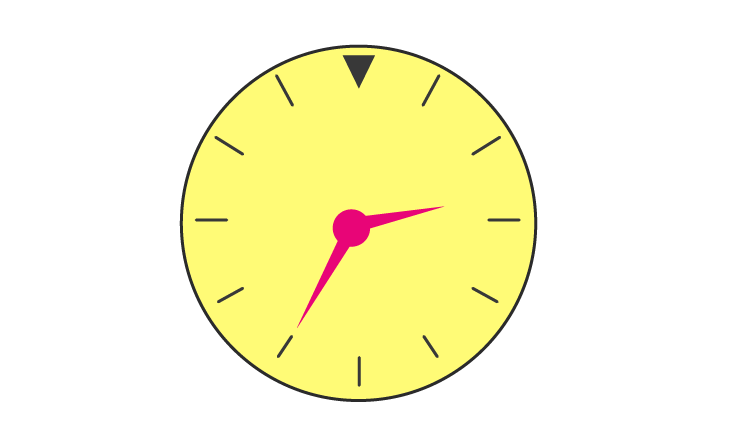a) 2.25

b) 2.35

c) 6.45

d) 9.25

The correct option is d) 9.25

Page No: 178

Q1. Name the spherical mirror which has:

a) virtual principal axis

b) real principal axis

a) Convex mirror

b) Concave mirror

Q2. Out of convex mirror and concave mirror, whose focus is situated behind the mirror?

Convex mirror has its focus behind.

Q3. Find the focal length of a concave mirror whose radius of curvature is 32cm.

Radius of curvature, R = 32cm

Focal length, f = ?

We know that,

f = R/2

f = 32/2 = 16cm

Q4. If the focal length of a convex mirror is 25cm, what is its radius of curvature?

Focal length, f = 25cm

Radius of curvature, R = ?

We know that,

f = R/2

R = 2f

R = 50cm

Q5. Fill in the following blanks with suitable words:

a) Parallel rays of light are reflected by a concave mirror to a point called the ……..

b) The focal length of a concave mirror is the distance from the ……… to the mirror.

c) A concave mirror ….. rays of light whereas a convex mirror ……. rays of light.

d) For a concave mirror, parallel rays of light appear to diverge from a point called the ……

a) Principal focus

b) Principle focus

c) Converges and diverges

d) Principle focus

Q6. What is a spherical mirror? Distinguish between a concave mirror and a convex mirror.

When the hollow sphere of glass as a reflecting surface, it is known as a spherical mirror. There are two types of spherical mirrors: concave mirror and convex mirror.

Difference between concave mirror and convex mirror:

a) A concave mirror has a bent surface from which the reflection of light takes place while a convex mirror has a bulged out surface from which the reflection of light takes place.

b) Concave mirrors converge the parallel rays of light while convex mirrors diverge the parallel rays of light.

Q7. Name the two types of spherical mirrors. What type of mirror is represented by the:

a) back side of a shining steel spoon?

b) front side of a shining steel spoon?

Two types of spherical mirrors are concave mirror and convex mirror.

a) Convex mirror

b) Concave mirror

Q8. What is the relation between the focal length and radius of curvature of a spherical mirror? Calculate the focal length of a spherical mirror whose radius of curvature is 25cm.

The relation between the focal length and the radius of curvature of a spherical mirror is:

f = R/2.

Given,

R = 25cm

f = ?

We know that, f = R/2

Therefore, f = 12.5cm

Q9. Explain with a suitable diagram, how a concave mirror converges a parallel beam of light rays. Mark clearly the pole, focus, and centre of curvature of concave mirror in this diagram.

After reflection, all the parallel light rays converge at the principal focus as the concave mirror converges the parallel beam of light rays. This is the reason why it is known as converging mirror.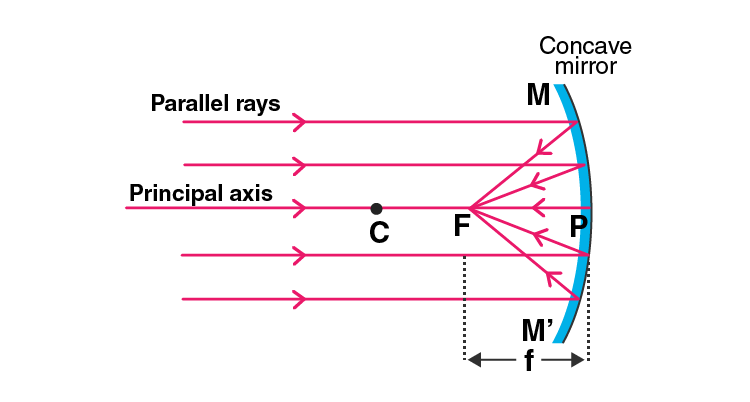Q10. Describe with a suitable diagram, how a convex mirror diverges a parallel beam of light rays. Mark clearly the pole, focus, and the centre of curvature of convex mirror in this diagram.

After the reflection, the light rays diverge from the principal focus and that is why convex mirror is also known as diverging mirror.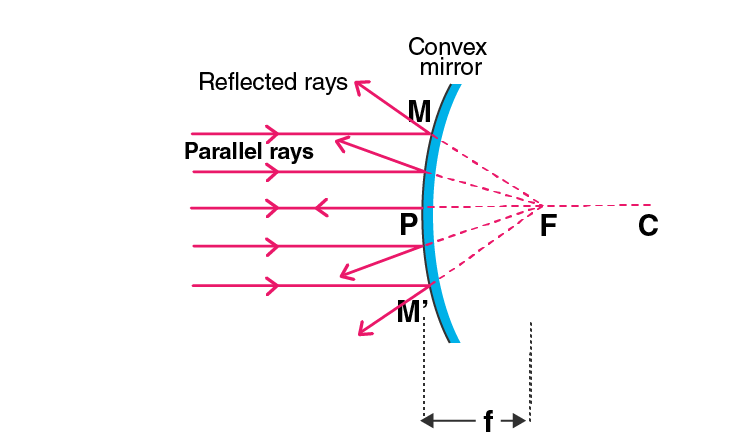Q11. Define a) centre of curvature

c) pole

d) principal axis

e) aperture of a spherical mirror with the help of a labelled diagram.

a) Centre of curvature: It is the centre of the hollow sphere of glass of which a mirror is a part.

b) Radius of curvature: It is the radius of the hollow sphere of glass of which a mirror is a part.

c) Pole: The centre of the spherical mirror.

d) Principal axis: It is the straight line that passes through the centre of curvature and to the pole of the spherical mirror.

e) Aperture: The portion of a mirror from where the reflection of light takes place.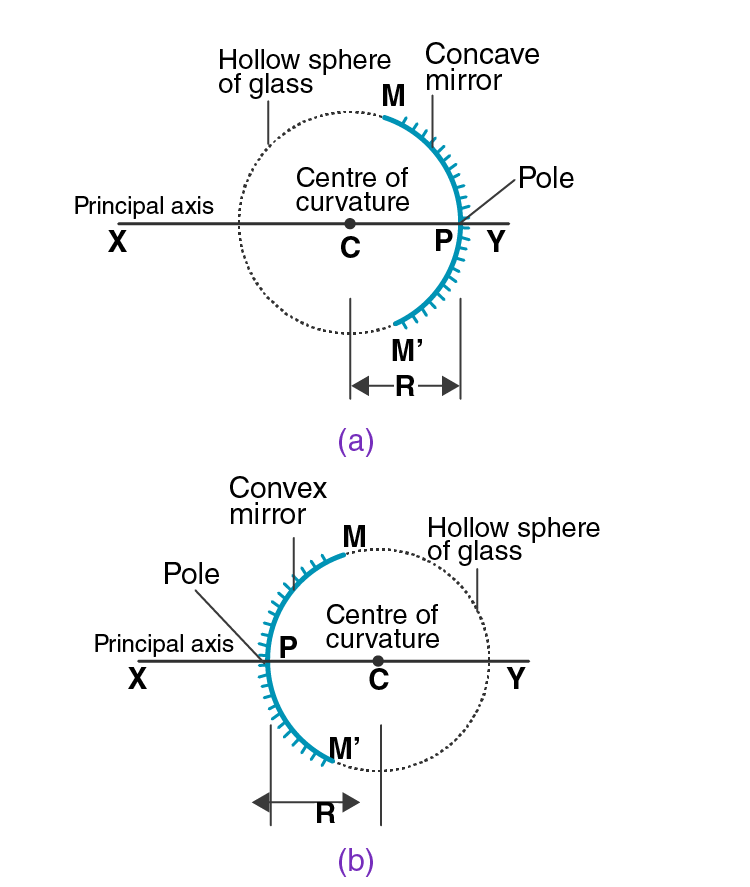Q12. a) Define i) principal focus of a concave mirror

ii) focal length of a concave mirror

b) Draw diagram to represent the action of a concave mirror on a beam of parallel light rays. Mark on this diagram principal axis, focus F, centre of curvature C, pole P, and the focal length f of the concave mirror.

a)

i) Principal focus of a concave mirror: The point on the principal axis on which all the light rays converge after reflection in a concave mirror.

ii) Focal length of a concave mirror: The distance between the pole and the principal axis is known as the focal length of a concave mirror.

b)Q13. a) What is meant by i) principal focus of a convex mirror ii) focal length of a convex mirror?

b) Draw diagram to show the action of convex mirror on a beam of parallel light rays. Mark on this diagram principal axis, focus F, centre of curvature C, pole P, and focal length f of the convex mirror.

a)

i) Principal focus of a convex mirror: When all the reflected light rays meet at a point after diverging in a convex mirror is known as principal focus of a convex mirror.

ii) Focal length of a convex mirror: The distance from the pole and the principal focus is known as the focal length of a convex mirror.

b)Multiple Choice Questions

Q14. In a convex spherical mirror, reflection of light takes place at:

a) a flat surface

b) a bent-in surface

c) a bulging-out surface

d) an uneven surface

The correct option is c) a bulging-out surface

Q15. A diverging mirror is:

a) a plane mirror

b) a convex mirror

c) a concave mirror

d) a shaving mirror

The correct option is b) a convex mirror

Q16. If R is the radius of curvature of a spherical mirror and f is its focal length then:

a) R = f

b) R = 2f

c) R = f/2

d) R = 3f

The correct option is b) R = 2f

Q17. The focal length of a spherical mirror of radius of curvature 30cm is:

a) 10cm

b) 15cm

c) 20cm

d) 30cm

The correct option is b) 15cm

Q18. If the focal length of a spherical mirror is 12.5cm less cm, its radius of curvature will be:

a) 25cm

b) 15cm

c) 20cm

d) 35cm

The correct option is a) 25cm

Page No: 189

Q1. For what position of an object, a concave mirror forms a real image equal in size to the object?

At the centre of curvature

Q2. Where should an object be placed in front of the concave mirror so as to obtain its virtual, erect, and magnified image?

The object should be placed between the pole and the focus.

Q3. For which positions of the object does a concave mirror produce an inverted, magnified, and real image?

When the object is placed between the focus and the centre of curvature.

Q4. If an object is placed at the focus of a concave mirror, where is the image formed?

The image is obtained at infinity.

Q5. If an object is at infinity in front of a concave mirror, where is the image formed?

The image is formed at the focus.

Q6. For what position of an object, a real and diminished image is formed by a concave mirror?

When the object is beyond the centre of curvature.

Q7. Copy this figure in your answer book and show the direction of the light ray after reflection: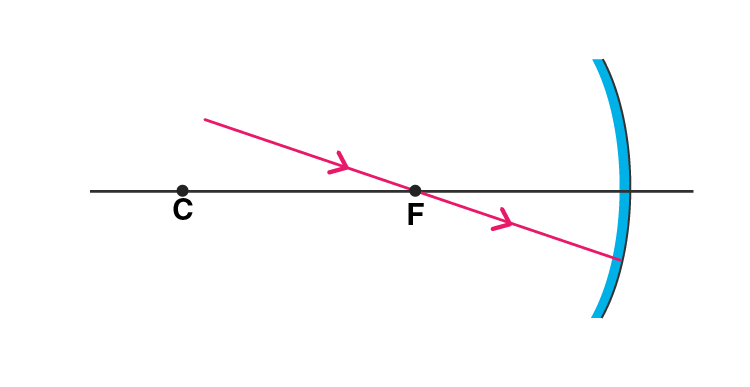Following is the direction of the light ray after reflection: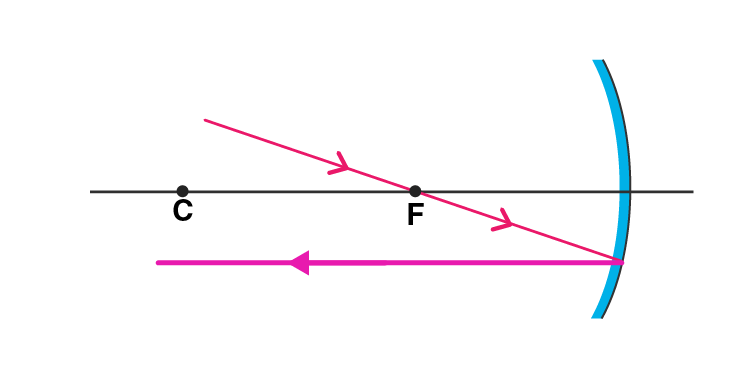Q8. Draw the following diagram in your answer book and show the formation of image of the object AB.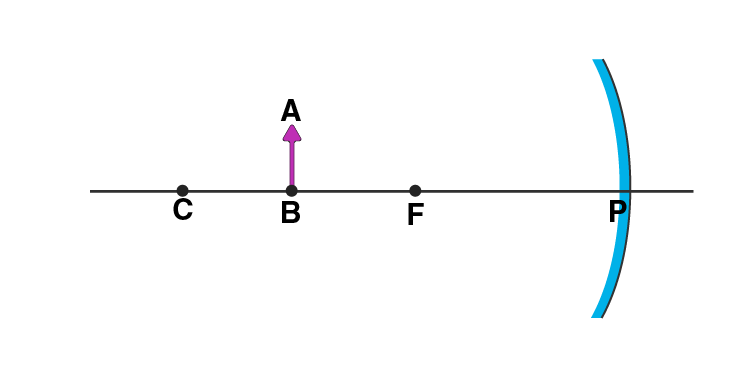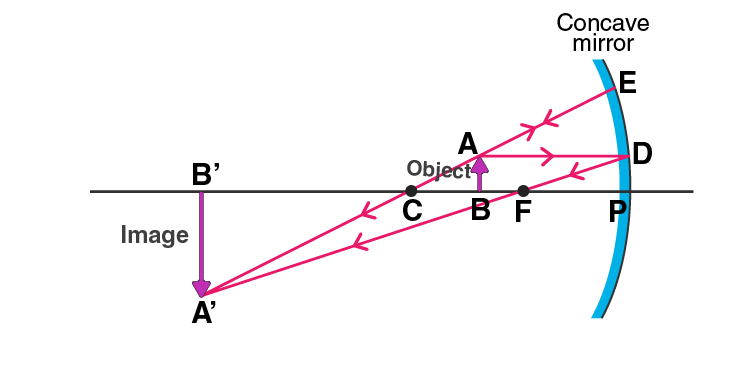Q9. Draw the following diagram in your answer book and show the formation of image with the help of suitable rays.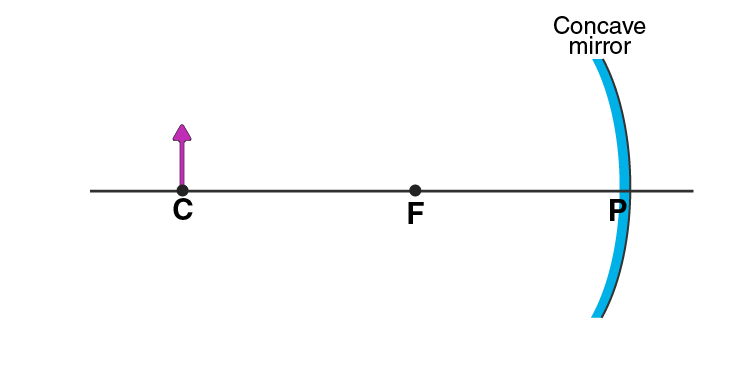Q10. Which type of mirror could be used as a dentist’s mirror?

A concave mirror can be used as a dentist mirror.

Q11. Which kind of mirror is used in the headlights of a car? Why is it used for this purpose?

Concave mirror is used in the headlights of a car. Concave mirror is used because when the light bulb is placed at the focus of a concave mirror reflector, the light rays diverge and gets collected by the concave reflector which is then reflected back as strong and parallel-sided beam of light.

Q12. Explain why a ray of light passing through the centre of curvature of a concave mirror gets reflected back along the same path.

A ray of light passing through the centre of curvature of a concave mirror gets reflected back along the same path because the angle between the angle of incidence and the angle of reflection are at 0 degrees as the light rays strike the surface at right angle.

Q13. What is the minimum number of rays required for locating the image formed by a concave mirror for an object? Draw a ray diagram to show the formation of a virtual image by a concave mirror.

For locating the image formed by a concave mirror, minimum two rays are required. Following is the ray diagram for the formation of a virtual image by a concave mirror: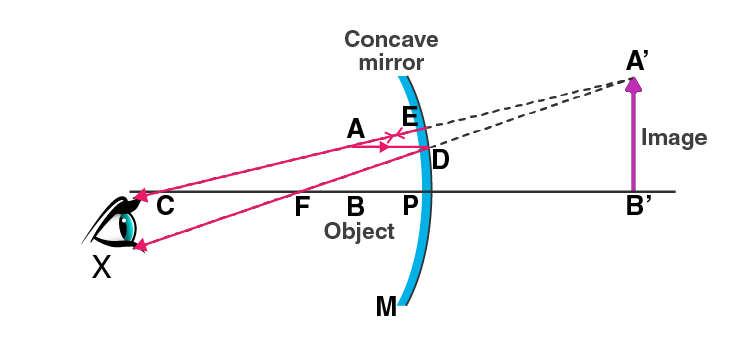Q14. With the help of a ray diagram, determine the position, nature, and the size of the image formed of an object placed at the centre of curvature of a concave mirror.

When an object is placed at the centre of curvature, C of a concave mirror, following is the image formed:

a) at the centre of curvature

b) real and inverted

c) the size of the image is equal to the size of an object

Q15. Describe with the help of a diagram, the nature, size, and the position of the image formed when an object is placed beyond the centre of curvature of a concave mirror.

When an object is placed beyond the centre of curvature of a concave mirror, the image formed is:

a) between the focus and the centre of curvature

b) real and inverted

c) smaller than the object

Q16. If an object is placed at a distance of 8cm from a concave mirror of focal length 10cm, discuss the nature of the image formed by drawing the ray diagram.

The focal length of the mirror, f = 10cm

The object is placed at = 8cm

Therefore, it can be said that the object is between the pole and the focus of the concave mirror

Therefore, the image formed is virtual, erect, and magnified.

Q17. Draw a ray diagram showing how a concave mirror can be used to produce a real, inverted, and diminished image of an object.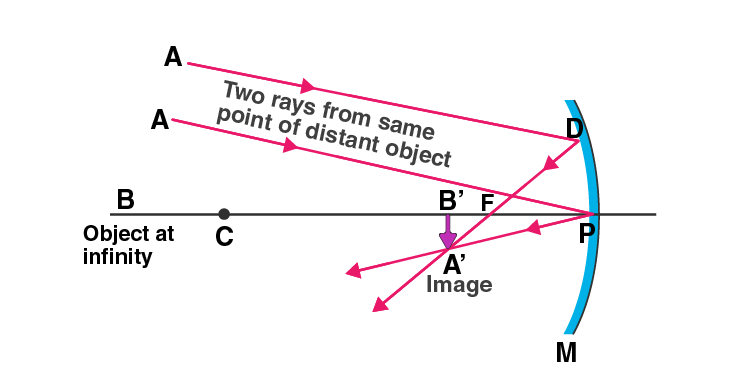Q18. Which mirror is used as torch reflector? Draw a labelled diagram to show how a torch reflector can be used to produce a parallel beam of light. Where is the bulb placed in relation to the torch reflector?

Concave mirror is used for a torch reflector.

Q19. State where an object must be placed so that the image formed by a concave mirror is:

a) erect and virtual

b) at infinity

c) the same size as the object

a) For erect and virtual, between the pole and the focus of the mirror

b) For infinity, at the focus of the mirror

c) For the same size as the object, at the centre of curvature of the mirror

Q20. With the help of a labelled ray diagram, describe how a converging mirror can be used to give an enlarged upright image of an object.

For obtaining an enlarged image which is upright, the object must be placed between the focus and the pole of the concave mirror.

In the diagram below, AD is drawn parallel to the principal axis such that D gets reflected and passes through the focus F. The second ray AE is passing through the centre of curvature striking at E and getting reflected back. DF and EC are the two diverging rays that do not intersect each other on the left side. DF and EC are produced backwards. When they are produced backward, they appear to intersect at the point A’. Therefore, A’ is the virtual image of point A. An enlarged image is obtained by A’B’ is drawn perpendicular to the axis.Q21. Make labelled ray diagrams to illustrate the formation of:

a) a real image by a converging mirror

b) a virtual image by a converging mirror

Mark clearly the pole, focus, centre of curvature, and the position of object in each case.

a) A real image by a converging mirror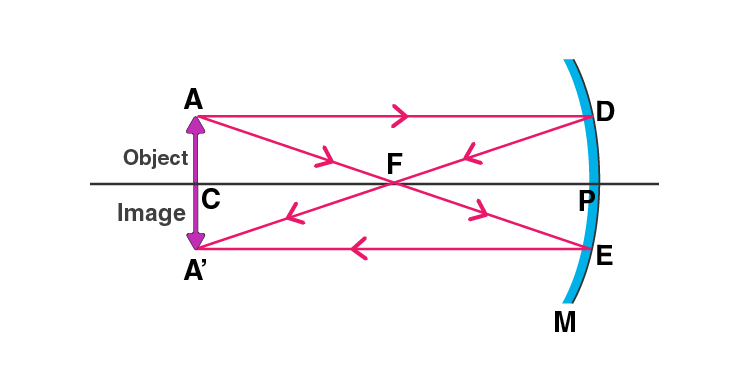b) A virtual image by a converging mirrorQ22. Briefly describe how you would find the focal length of a concave mirror quickly but approximately.

For determining the focal length of a concave mirror, the object should be placed a considerable distance from the concave mirror such that the image is formed at the focus. This is done by focusing a distant object such as a tree by using a concave mirror. A sharp image of the tree is formed at the focus of the concave mirror. Therefore, the distance of the image from the concave mirror will be equal to the focal length of the concave mirror.

Q23. Which type of mirror is used in a solar furnace? Support your answer with reason.

A concave mirror is used in a solar furnace. The solar furnace is placed at the focus of a large concave reflector. When the light rays fall on the surface of the concave mirror, the rays get reflected and meet at the focus of the mirror due to the converging nature of the concave mirror resulting in the heating of the furnace.

Q24. Name the type of mirror used by dentists. How does it help?

Dentist use a concave mirror. Dentist hold the concave mirror in such a way that the tooth lies within the focus. A magnified image of the tooth is seen by the dentist which helps them in examining the defects.

Q25. Explain why concave mirrors are used as shaving mirrors.

Concave mirrors are used as a shaving mirrors because when the face is placed close to the mirror, a magnified image is obtained which is erect in nature. Thus helping in smoother shave.

Q26. Give two uses of concave mirrors. Explain why you would choose concave mirrors for these uses.

Following are the uses of a concave mirrors:

a) Concave mirrors are used as a shaving mirror as a magnified and erect image is formed helping in smooth shave.

b) Concave mirrors are also used by the dentists as an enlarged image is obtained helping them in detecting the defects in the tooth.

Q27. a) Draw ray-diagrams to show the formation of images when the object is placed in front of a concave mirror:

i) between its pole and focus

ii) between its centre of curvature and focus describe the nature, size, and position of the image formed in each case

b) State one use of concave mirror based on the formation of images as in case (z) above.

a)

i) When the object is placed between the pole and the focus of a concave mirror, the image formed is behind the mirror and it is virtual, erect, and larger than the object.ii) When the object is placed between the centre of curvature and focus of a concave mirror, the image formed is beyond the centre of curvature. The image obtained is real, inverted, and larger than the object.b) One use of concave mirror based on the formations of images is it is used as a shaving mirror.

Q28. a) Give two circumstances in which a concave mirror can form a magnified image of an object placed in front of it. Illustrate your answer by drawing labelled ray diagrams for both.

b) Which one of these circumstances enables a concave mirror to be used as shaving mirror?

a)

i) When the object is placed between the pole and the focus of a concave mirror, a magnified image is formed.ii) When the object is placed between the focus and the centre of curvature of a concave mirror, a magnified image is formed.b) A concave mirror can be used as a shaving mirror when the object is placed between the pole and the focus of the concave mirror.

Multiple Choice Questions

Q29. The real image formed by a concave mirror is larger than the object when the object is:

a) at a distance equal to radius of curvature

b) at a distance less than the focal length

c) between focus and centre of curvature

d) at a distance greater than radius of curvature

The correct option is c) between focus and centre of curvature

Q30. The real image formed by a concave mirror is smaller than the object if the object is:

a) between centre of curvature and focus

b) at a distance greater than radius of curvature

c) at a distance equal to radius of curvature

d) at a distance equal to focal length

The correct option is b) at a distance greater than radius of curvature

Q31. The image formed by a concave mirror is virtual, erect, and magnitude. The position of object is:

a) at focus

b) between focus and centre of curvature

c) at pole

d) between pole and focus

The correct option is d) between pole and focus

Q32. The image formed by a concave mirror is real, inverted, and of the same size as the object. The position of the object must then be:

a) at the focus

b) between the centre of curvature and focus

c) at the centre of curvature

d) beyond the centre of curvature

The correct option is c) at the centre of curvature

Q33. The image formed by a concave mirror is real, inverted, and highly diminished (much smaller than the object). The object must be:

a) between pole and focus

b) at focus

c) at the centre of curvature

d) at infinity

The correct option is d) at infinity

Q34. The angle of incidence for a ray of light passing through the centre of curvature of a concave mirror is:

a) 45 degree

b) 90 degree

c) 0 degree

d) 180 degree

The correct option is c) 0 degree

Q35. In the concave reflector of a torch, the bulb is placed:

a) between the pole and focus of reflector

b) at the focus of reflector

c) between focus and centre of curvature of reflector

d) at the centre of curvature of reflector

The correct option is b) at the focus of reflector

Q36. The focal length of a small concave mirror is 2.5cm. In order to use this concave mirror as a dentist’s mirror, the distance of tooth from the mirror should be:

a) 2.5cm

b) 1.5cm

c) 4.5cm

d) 3.5cm